Jekyll2022-04-15T00:15:59-04:00https://www.alexejgossmann.com/feed.xml0-fold Cross-ValidationBlogging aspirations: machine learning, statistics, deep learning, math, theory, application, coding, creativity. Alexej Gossmann0foldcv@pm.meConcordance Correlation Coefficient2020-01-06T00:00:00-05:002020-01-06T00:00:00-05:00https://www.alexejgossmann.com/cccIf we collect $$n$$ independent pairs of observations $$(y_{11}, y_{12}), (y_{21}, y_{22}), \dots, (y_{n1}, y_{n2})$$ from some bivariate distribution, then how can we estimate the expected squared perpendicular distance of each such point in the 2D plane from the 45-degree line?

Assume that the random two-dimensional vector $$(Y_1, Y_2)$$ follows a bivariate distribution with mean $$\E(Y_1, Y_2) = (\mu_1, \mu_2)$$, and covariance matrix with entries $$\mathrm{Var}(Y_1) = \sigma_1^2$$, $$\mathrm{Var}(Y_2) = \sigma_2^2$$ and $$\mathrm{Cov}(Y_1, Y_2) = \sigma_{12}$$.

The squared perpendicular distance of the random point $$(Y_1, Y_2)$$ from the 45-degree line is

$\begin{equation*} D^2 = \frac{(Y_1 - Y_2)^2}{2}, \end{equation*}$

see the figure below. Thus, the expected value of the squared perpendicular distance times two (for notational convenience) is given by,

\begin{align} \E\left[ 2D^2 \right] &= \E\left[ (Y_1 - Y_2)^2 \right] \nonumber \\ &= \E\left[ \left( (Y_1-\mu_1) - (Y_2-\mu_2) + \mu_1-\mu_2 \right)^2 \right] \nonumber \\ &= \E\left[ \left((Y_1-\mu_1) - (Y_2-\mu_2) \right)^2 \right] + (\mu_1-\mu_2)^2 \nonumber \\ &= (\mu_1-\mu_2)^2 + \sigma_1^2 + \sigma_2^2 - 2\sigma_{12} \label{eq:decomp1} \\ &= (\mu_1-\mu_2)^2 + (\sigma_1 - \sigma_2)^2 + 2[1 - \rho] \sigma_1 \sigma_2. \nonumber \end{align}

To answer the question raised above, we can estimate the value of equation $$\eqref{eq:decomp1}$$ based on $$n$$ pairs of observations $$(y_{11}, y_{12}), (y_{21}, y_{22}), \dots, (y_{n1}, y_{n2})$$ substituting the respective sample mean, sample variance, and covariance estimates for $$\mu_1, \mu_2, \sigma_1^2, \sigma_2^2, \sigma_{12}$$ respectively.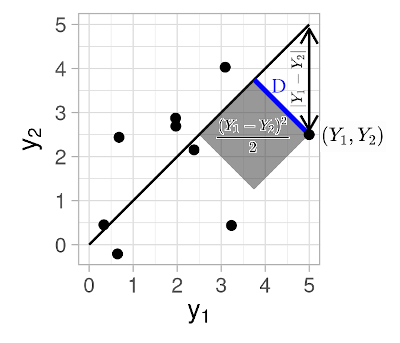## Defining the Concordance Correlation Coefficient

That’s great, but why should we spend any time thinking about the expected distance from the 45-degree line? What’s interesting about it?

Apart from delighting in the pure joy of doing mathematics and taking pleasure in the experience of mathematical beauty… :joy: :stuck_out_tongue_closed_eyes: … A measure of distance from the 45-degree line naturally quantifies the (dis)agreement between the two sets of observations. For example, we may have measured the same target entities using two different measurement instruments, and may want to know if and to what extent they agree.

Towards quantifying the extent of the (dis)agreement between two sets of observations it is natural to try to scale (or normalize) the quantity of equation $$\eqref{eq:decomp1}$$ to the range $$[0, 1]$$. However, it turns out that, rather than scaling to a $$[0, 1]$$ range, it is customary to scale this quantity to the range from -1 to 1 as follows,

$\begin{equation} \mathrm{CCC} := 1 - \frac{\E\left[ (Y_1 - Y_2)^2 \right]}{(\mu_1-\mu_2)^2 + \sigma_1^2 + \sigma_2^2} = \frac{2\sigma_{12}}{(\mu_1-\mu_2)^2 + \sigma_1^2 + \sigma_2^2}. \label{eq:ccc} \end{equation}$

This expression, first introduced by (Lin, 1989), is known as the Concordance Correlation Coefficient, abbreviated as CCC hereafter.

## Concordance Correlation Coefficient vs. Pearson correlation coefficient

The scaling into the range from -1 to 1 may have been motivated by the fact that the Pearson correlation coefficient $$\rho$$ also falls within the $$[-1, 1]$$ range. In fact, analogous to how a Pearson correlation coefficient $$\rho=1$$ signifies perfect positive correlation, a CCC of 1 designates that the paired observations fall exactly on the line of perfect concordance (i.e., the 45-degree diagonal line).

Further aspects of the relationship to the Pearson correlation coefficient $$\rho$$ become visible if we rewrite the CCC further into the following set of equations.

$\begin{equation} \mathrm{CCC} = \rho C, \label{eq:ccc2} \end{equation}$

where

$\begin{equation} C = \frac{2}{v + \frac{1}{v} + u^2}, v = \frac{\sigma_1}{\sigma_2}, u = \frac{(\mu_1 - \mu_2)^2}{\sigma_1 \sigma_2}. \label{eq:c} \end{equation}$

From equations $$\eqref{eq:ccc2}$$ and $$\eqref{eq:c}$$ we observe that:

• CCC always has the same sign as $$\rho$$.
• CCC is 0 if and only if $$\rho = 0$$ (with exception of cases when $$\rho$$ is undefined but CCC can still be computed via equation $$\eqref{eq:ccc}$$).
• We can consider $$\rho$$ to be a measure of precision, i.e., how far each point deviates from the best fitting line. Thus, CCC combines $$\rho$$ (as a measure of precision) with an additional measure of accuracy denoted by $$C$$ in equations $$\eqref{eq:ccc2}$$ and $$\eqref{eq:c}$$, whereby:
• $$C$$ quantifies how far the best-fit line deviates from the 45-degree line.
• When the data lie exactly on the 45-degree line, then we have that C = 1. The further the observations deviate from the 45-degree line, the further C is from 1 and the closer it is to 0.
• In particular, $$v$$ from equation $$\eqref{eq:c}$$ can be considered a measure of scale shift, and $$u$$ from equation $$\eqref{eq:c}$$ a measure of location shift relative to the scale.

Now it turns out that the Pearson correlation coefficient $$\rho$$ has one major shortcoming when assessing reproducibility of measurements, such as when comparing two instruments that measure the same target entity.

:point_right: Unlike CCC, $$\rho$$ is invariant to additive or multiplicative shifts by a constant value, referred to as location shift and scale shift respectively in the following set of figures: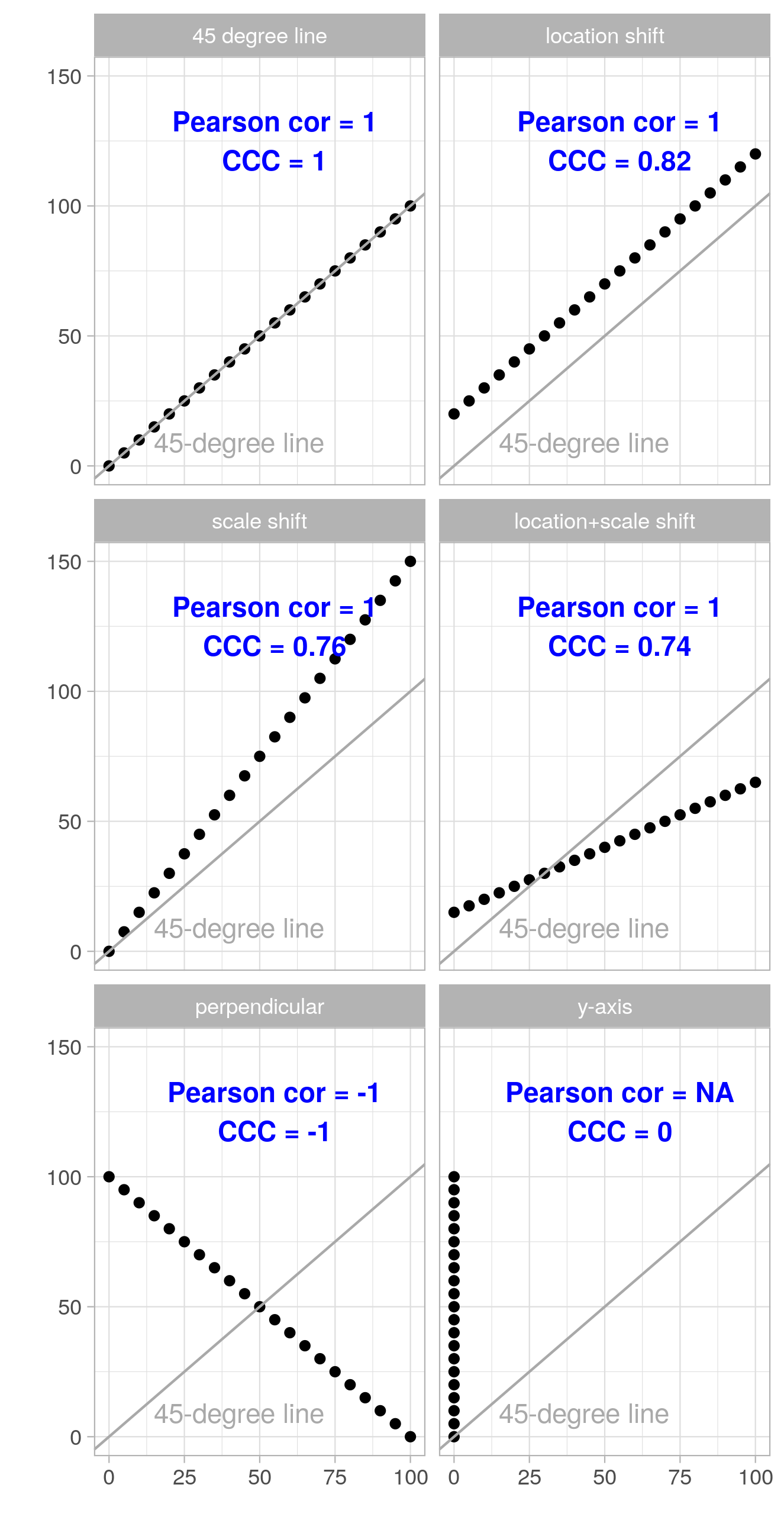Looking at the above figures we see that the magnitude of the Pearson correlation coefficient $$\rho$$ does not change under location and scale shift (though the sign may flip). The CCC on the other hand quantifies the deviation from the 45-degree line, which is due to location and scale shifts in these examples, rather well.

This makes the CCC a better metric when we want to assess how well one measurement can reproduce another (i.e., how close the measurement pairs fall to the 45-degree line), while we would use $$\rho$$ if what we want is quantifying to what extent the measurement pairs can be described by a linear equation (with any intercept and slope).

The following figures show the same examples where both the $$x$$ and the $$y$$ coordinates are augmented with Gaussian noise (mean 0, standard deviation 15; the same realization of the random noise is used within each subfigure). We see that both $$\rho$$ and CCC move further away from the extreme values of $$-1$$, $$0$$, and $$1$$ as noise is added.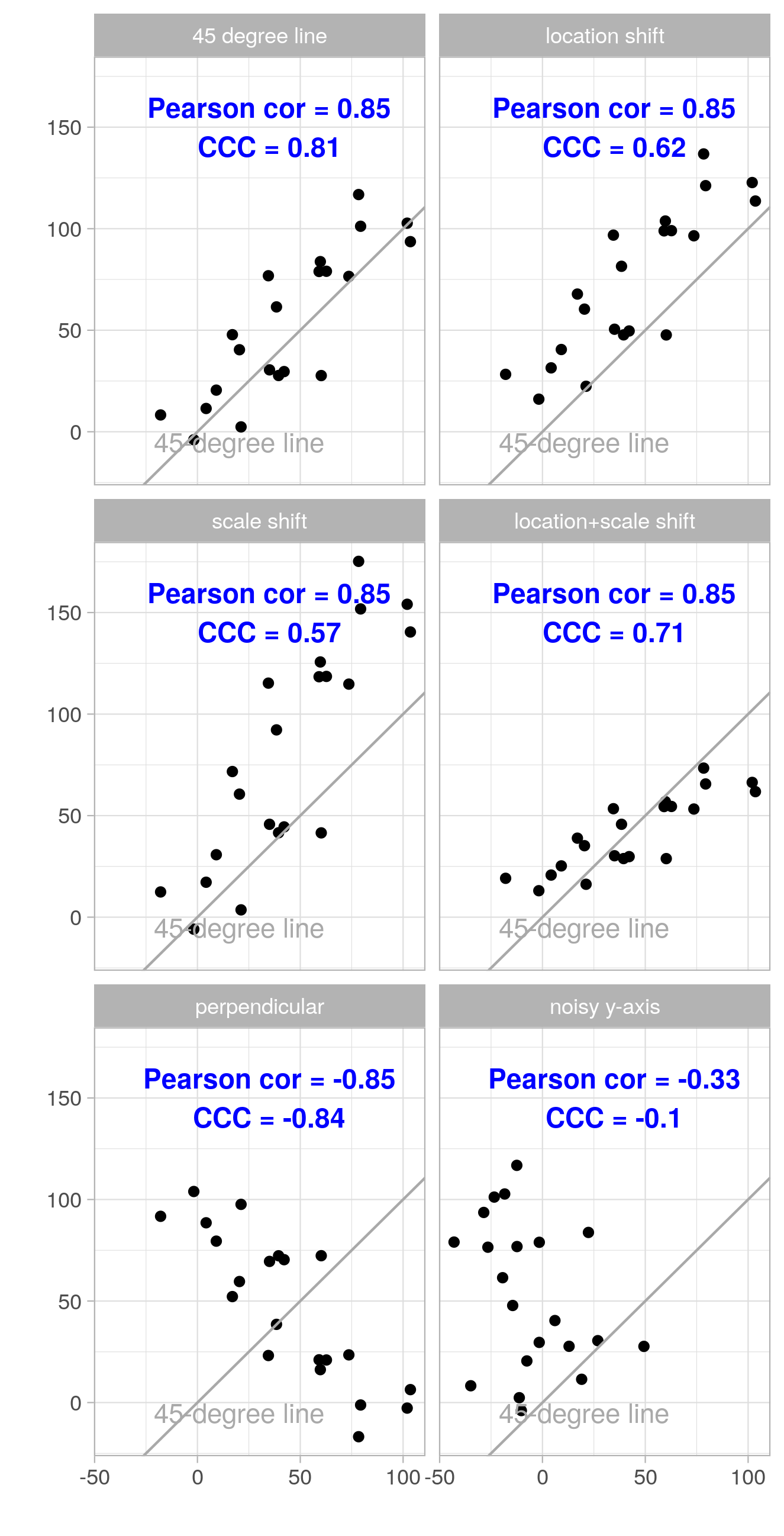## What’s CCC good for? Reproducibility?

As hinted above, you may want to compare two instruments that aim to measure the same target entity, or two assays that aim to measure the same analyte, or other quantitative measurement procedures or devices. For example, one set of measurements may be obtained by what’s considered the “gold standard”, while the other set of measurements may be collected by a new instrument/assay/device that may be cheaper or in some other way preferable to the “gold standard” instrument/assay/device. Then one would wish to demonstrate that the collected two sets of measurements are equivalent. (Lin, 1989) refers to this type of agreement or similarity between two sets of measurements as reproducibility of measurements. The paper considers the following two illustrative examples:

(1) Can a “Portable $ave” machine (actual name withheld) reproduce a gold-standard machine in measuring total bilirubin in blood? (2) Can an in-vitro assay for screening the toxicity of biomaterials reproduce from trial to trial? And indeed this type of reproducibility assessment is a task where CCC has some clear advantages over the Pearson correlation coefficient, as seen in the figures above, as well as over some other approaches, as discussed in (Lin, 1989) in detail. A couple of shortcomings of common statistical approaches (when applied to the reproducibility assessment problem in question) are the following: • The paired t-test can reject a highly reproducible set of measurements when the variance is very small, while it fails to detect poor agreement in pairs of data when the means are equal. • The least squares approach of testing intercept equal to 0 and slope equal to 1 fails to detect nearly perfect agreement when the residual errors are very small, while it has increasingly little chance to reject the null hypothesis of agreement the more the data are scattered. I will end here. However, if you want to go deeper into the topic I invite you to check out the original paper by Lin for a more thorough discussion of the merits of the CCC as well as for its statistical properties. Moreover, since the publication of (Lin, 1989) there of course has been follow-up work, which I didn’t read (so, I may update this blog post in the future). 1. Lin, L. I. (1989). A concordance correlation coefficient to evaluate reproducibility. Biometrics, 45(1), 255–268. https://www.ncbi.nlm.nih.gov/pubmed/2720055 ]]> From conditional probability to conditional distribution to conditional expectation, and back2018-08-12T00:00:00-04:002018-08-12T00:00:00-04:00https://www.alexejgossmann.com/conditional_distributionsI can’t count how many times I have looked up the formal (measure theoretic) definitions of conditional probability distribution or conditional expectation (even though it’s not that hard :weary:) Another such occasion was yesterday. This time I took some notes. ## From conditional probability → to conditional distribution → to conditional expectation Let $$X$$ and $$Y$$ be two real-valued random variables. ### Conditional probability For a fixed set $$B$$ (Feller, 1966, p. 157) defines conditional probability of an event $$\{Y \in B\}$$ for given $$X$$ as follows. By $$\prob(Y \in B \vert X)$$ (in words, “a conditional probability of the event $$\{Y \in B\}$$ for given $$X$$”) is meant a function $$q(X, B)$$ such that for every set $$A \in \mathbb{R}$$ $\prob(X \in A, Y \in B) = \int_A q(x, B) \mu(dx)$ where $$\mu$$ is the marginal distribution of $$X$$. (where $$A$$ and $$B$$ are both Borel sets on $$\R$$.) That is, the conditional probability can be defined as something that, when integrated with respect to the marginal distribution of $$X$$, results in the joint probability of $$X$$ and $$Y$$. Moreover, note that if $$A = \R$$ then the above formula yields $$\prob(Y \in B)$$, the marginal probability of the event $$\{ Y \in B \}$$. #### Example For example, if the joint distribution of two random variables $$X$$ and $$Y$$ is the following bivariate normal distribution $\begin{pmatrix} X \\ Y \end{pmatrix} \sim \mathcal{N} \left( \begin{pmatrix} \mu_X \\ \mu_Y \end{pmatrix}, \begin{pmatrix} \sigma^2_X & \rho \sigma_X \sigma_Y \\ \rho \sigma_X \sigma_Y & \sigma^2_Y \end{pmatrix} \right),$ then by sitting down with a pen and paper for some amount of time, it is not hard to verify that the function $q(x, B) = \int_B \frac{1}{\sqrt{2\pi(1-\rho^2)}\sigma_Y} \exp\left(-\frac{\left(y - \mu_Y+\frac{\sigma_Y}{\sigma_X}\rho( x - \mu_X)\right)^2}{2(1-\rho^2)\sigma_Y^2}\right) \mathrm{d}y$ in this case satisfies the above definition of $$\prob(Y \in B \vert X)$$. ### Conditional distribution Later on (Feller, 1966, p. 159) follows up with the notion of conditional probability distribution: By a conditional probability distribution of $$Y$$ for given $$X$$ is meant a function $$q$$ of two variables, a point $$x$$ and a set $$B$$, such that 1. for a fixed set $$B$$ $q(X, B) = \prob(Y \in B \vert X )$ is a conditional probability of the event $$\{Y \in B\}$$ for given $$X$$. 2. $$q$$ is for each $$x$$ a probability distribution. It is also pointed out that In effect a conditional probability distribution is a family of ordinary probability distributions and so the whole theory carries over without change. (Feller, 1966) When I first came across this viewpoint, I found it incredibly enlightening to regard the conditional probability distribution as a family of ordinary probability distributions. :smile: #### Example For example, assume that $$X$$ is an integer-valued and non-negative random variable, and that the conditional probability distribution of $$Y$$ for given $$X$$ is an F-distribution (denoted $$\mathrm{F}(d_1, d_2)$$) with $$d_1 = e^X$$ and $$d_2 = 2^X$$ degrees of freedom. Then the conditional probability distribution of $$(Y \vert X)$$ can be regarded as a family of probability distributions $$\mathrm{F}(e^x, 2^x)$$ for $$x = 0, 1, 2, \dots$$, whose probability density functions look like this: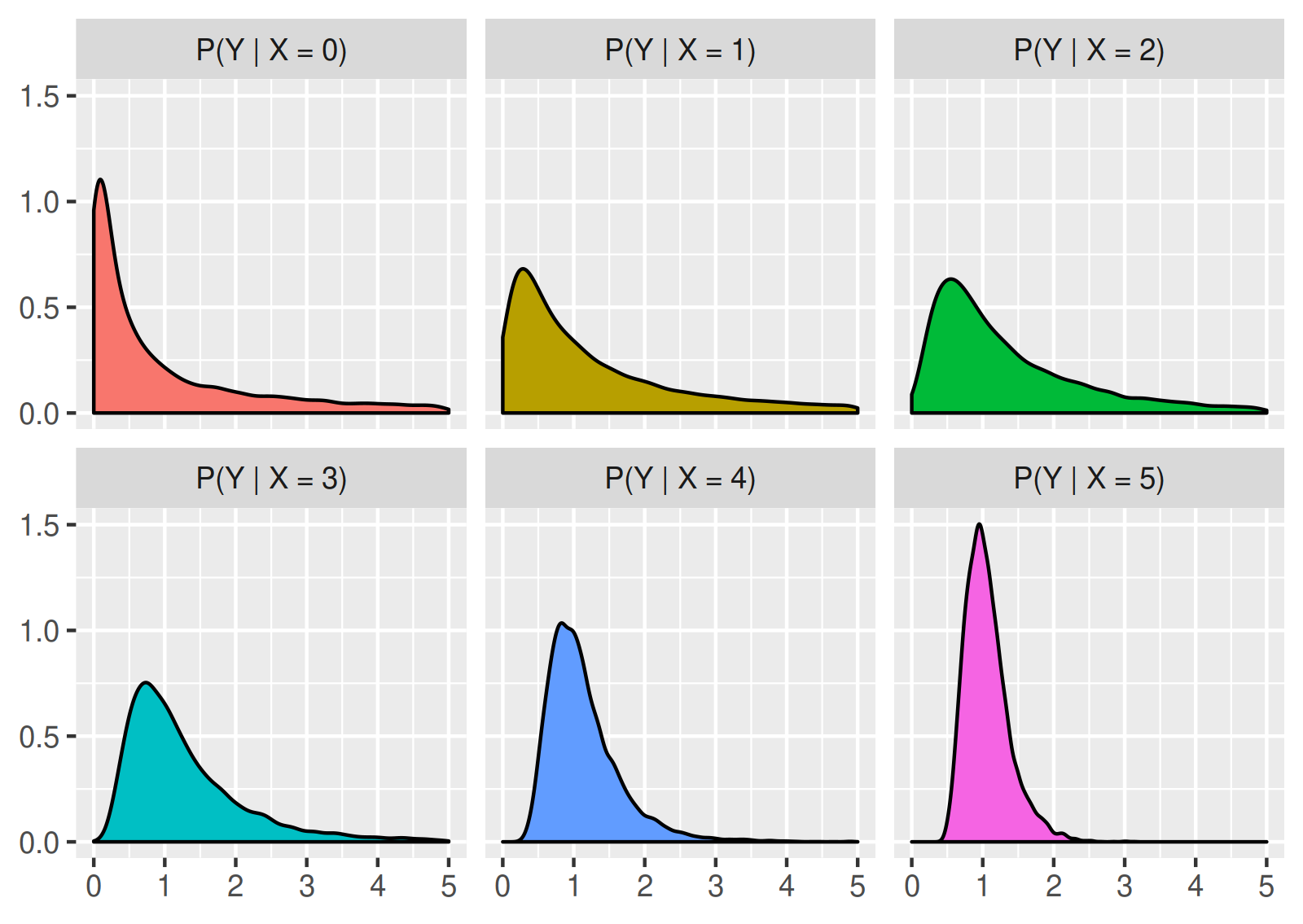In addition, as pointed out above, if we know the marginal distribution of $$X$$, then the conditional probability distribution of $$(Y \vert X)$$ can be used to obtain the marginal probability distribution of $$Y$$, or to randomly sample from the marginal distribution. Practically it means that if we randomly generate a value of $$X$$ according to its probability distribution, and use this value to randomly generate a value of $$Y$$ according to the conditional distribution of $$Y$$ for the given $$X$$, then the observations resulting from this procedure follow the marginal distribution of $$Y$$. Continuing the previous example, assume that $$X$$ follows a binomial distribution with parameters $$n = 5$$ and $$p = 0.5$$. Then the described simulation procedure estimates the following shape for the probability density function of $$\prob(Y)$$, the marginal distribution of $$Y$$: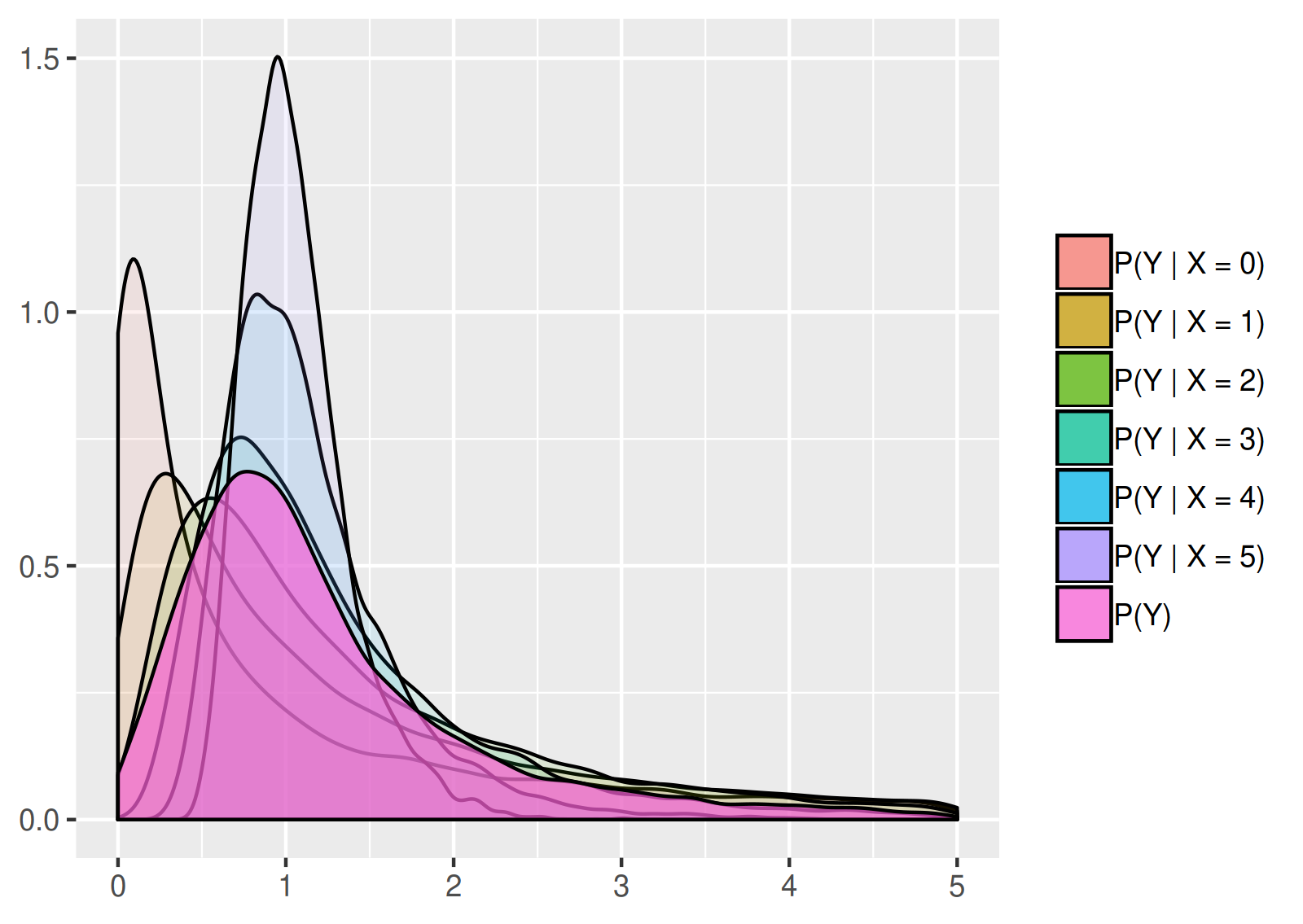### Conditional expectation Finally, (Feller, 1966, p. 159) introduces the notion of conditional expectation. By the above, for given a value $$x$$ we have that $q(x, B) = \prob(Y \in B \vert X = x), \quad\forall B\in\mathcal{B}$ (here $$\mathcal{B}$$ denotes the Borel $$\sigma$$-algebra on $$\R$$), and therefore, a conditional probability distribution can be viewed as a family of ordinary probability distributions (represented by $$q$$ for different $$x$$s). Thus, as (Feller, 1966, p. 159) points out, if $$q$$ is given then the conditional expectation “introduces a new notation rather than a new concept.” A conditional expectation $$E(Y \vert X)$$ is a function of $$X$$ assuming at $$x$$ the value $\E(Y \vert X = x) = \int_{-\infty}^{\infty} y q(x, dy)$ provided the integral converges. Note that, because $$\E(Y \vert X)$$ is a function of $$X$$, it is a random variable, whose value at an individual point $$x$$ is given by the above definition. Moreover, from the above definitions of conditional probability and conditional expectation it follows that $\E(Y) = \E(\E(Y \vert X)).$ #### Example [cont.] We continue with the last example. From the properties of the F-distribution we know that under this example’s assumptions on the conditional distribution, it holds that $\E(Y \vert X = x) = \begin{cases} \frac{d_2}{d_2 - 2} = \frac{2^x}{2^x - 2}, \quad x > 1,\\ \infty, \quad x \leq 1. \end{cases}$ A rather boring strictly decreasing function of $$x$$ converging to $$1$$ as $$x\to\infty$$. Thus, under the example’s assumption on the distribution of $$X$$, the conditional expectation $$\E(Y \vert X)$$ is a discrete random variable, which has non-zero probability mass at the values $$2, 4/3, 8/7, 16/15,$$ and $$\infty$$. ## From conditional expectation → to conditional probability An alternative approach is to define the conditional expectation first, and then to define conditional probability as the conditional expectation of the indicator function. This approach seems less intuitive to me. However, it is more flexible and more general, as we see below. ### Conditional expectation #### A definition in 2D Let $$X$$ and $$Y$$ be two real-valued random variables, and let $$\mathcal{B}$$ denote the Borel $$\sigma$$-algebra on $$\R$$. Recall that $$X$$ and $$Y$$ can be represented as mappings $$X: \Omega \to \R$$ and $$Y: \Omega \to \R$$ over some measure space $$(\Omega, \mathcal{A}, \prob)$$. We can define $$\mathrm{E}(Y \vert X=x)$$, the conditional expectation of $$Y$$ given $$X=x$$, as follows. A $$\mathcal{B}$$-measurable function $$g(x)$$ is the conditional expectation of $$Y$$ for given $$x$$, i.e., $\mathrm{E}(Y \vert X=x) = g(x),$ if for all sets $$B\in\mathcal{B}$$ it holds that $\int_{X^{-1}(B)} Y(\omega) d\prob(\omega) = \int_{B} g(x) d\prob^X(x),$ where $$\prob^X$$ is the marginal probability distribution of $$X$$. #### Interpretation in 2D If $$X$$ and $$Y$$ are real-valued one-dimensional, then the pair $$(X,Y)$$ can be viewed as a random vector in the plane. Each set $$\{X \in A\}$$ consists of parallels to the $$y$$-axis, and we can define a $$\sigma$$-algebra induced by $$X$$ as the collection of all sets $$\{X \in A\}$$ on the plane, where $$A$$ is a Borel set on the line. The collection of all such sets forms a $$\sigma$$-algebra $$\mathcal{A}$$ on the plane, which is contained in the $$\sigma$$-algebra of all Borel sets in $$\R^2$$. $$\mathcal{A}$$ is called the $$\sigma$$-algebra generated by the random variable $$X$$. Then $$\mathrm{E}(Y \vert X)$$ can be equivalently defined as a random variable such that $\mathrm{E}(Y\cdot I_{A}) = \mathrm{E}(\mathrm{E}(Y \vert X) \cdot I_{A}), \quad \forall A\in\mathcal{A},$ where $$I_{A}$$ denotes the indicator function of the set $$A$$. #### A more general definition of conditional expectation The last paragraph illustrates that one could generalize the definition of the conditional expectation of $$Y$$ given $$X$$ to the conditional expectation of $$Y$$ given an arbitrary $$\sigma$$-algebra $$\mathcal{B}$$ (not necessarily the $$\sigma$$-algebra generated by $$X$$). This leads to the following general definition, which is stated in (Feller, 1966, pp. 160-161) in a slightly different notation. Let $$Y$$ be a random variable, and let $$\mathcal{B}$$ be a $$\sigma$$-algebra of sets. 1. A random variable $$U$$ is called a conditional expectation of $$Y$$ relative to $$\mathcal{B}$$, or $$U = \E(Y \vert \mathcal{B})$$, if it is $$\mathcal{B}$$-measurable and $\E(Y\cdot I_{B}) = \E(U \cdot I_{B}), \quad \forall B\in\mathcal{B}.$ 2. If $$\mathcal{B}$$ is the $$\sigma$$-algebra generated by a random variable $$X$$, then $$\E(Y \vert X) = \E(Y \vert \mathcal{B})$$. ### Back to conditional probability and conditional distributions Let $$I_{\{Y \in A\}}$$ be a random variable that is equal to one if and only if $$Y\in A$$. The conditional probability of $$\{Y \in A\}$$ given $$X = x$$ can be defined in terms of a conditional expectation as $\prob(Y \in A \vert X = x) = \E(I_{\{Y \in A\}} \vert X = x).$ Under certain regularity conditions the above defines the conditional probability distribution of $$(Y \vert X)$$. ## References 1. Feller, W. (1966). An introduction to probability theory and its applications (Vol. 2). John Wiley & Sons. ]]> Alexej Gossmann Setting up an HTTPS static site using AWS S3 and Cloudfront (and also Jekyll and s3_website)2018-07-27T00:00:00-04:002018-07-27T00:00:00-04:00https://www.alexejgossmann.com/AWS_S3_and_CloudFrontFor a while now I wanted to migrate my websites away from Github pages. While Github provides an excellent free service, there are some limitations to its capabilities, and the longer I wait the harder (or the more inconvenient) it becomes to migrate away from gh-pages. AWS S3 + CloudFront is a widely-used alternative that has been around for a long time. Moreover, I was planning to get more familiar with AWS at all levels anyway. So, it’s a great learning opportunity too. There are a number of very helpful tutorials online on how to set up an HTTPS static site using AWS S3 and CloudFront. Of course, as always the case with blog articles, they may be outdated, incomplete, and generally not as trustworthy as the official AWS documentation on the topic, which is pretty good too; but it is also somewhat fragmented and inconvenient to follow. So I wrote my own summary to refer to in the future. ### 1 Set up a static site, yet without CloudFront and without HTTPS First, we set up a static HTTP site without a custom domain on AWS S3: • Create a bucket named example.com (obviously replace example.com with your own domain). • Follow the procedure given at https://docs.aws.amazon.com/AmazonS3/latest/dev/HostingWebsiteOnS3Setup.html to enable website hosting for the bucket, and to make it publicly readable; (optionally) if you want to understand the AWS bucket access policy language see https://docs.aws.amazon.com/AmazonS3/latest/dev//access-policy-language-overview.html, and follow the links from there. • Test the S3 website: Upload an index.html to the bucket (you can keep all options for the upload at their default values). Then go to http://example.com.s3-website-us-east-1.amazonaws.com/ (where you need to replace example.com with the bucket name, and us-east-1 with your bucket’s region), and see if the contents of index.html show up. Yay :laughing: we have a working website!! …without a custom domain or https yet :sweat_smile: The www subdomain: Now prepare another S3 bucket for the subdomain “www.example.com” to be later redirected to the root domain “example.com” (btw, if you so wish, www.example.com can be the main S3 bucket and the example.com bucket can be configured to redirect — just swap their roles in this entire writeup): • Create a bucket named www.example.com (all options can be left at their defaults; this bucket doesn’t need to be publicly readable). • Configure www.example.com to redirect all requests to example.com following Step 2.3 from the AWS docs at https://docs.aws.amazon.com/AmazonS3/latest/dev/website-hosting-custom-domain-walkthrough.html. • Test the endpoints for the redirect by going to http://www.example.com.s3-website-us-east-1.amazonaws.com/ (as before replace the bucket name and region accordingly). Map the domain and subdomain to their S3 buckets: Amazon Route 53 is a service that maintains a mapping between the alias records and the IP of the bucket. You need to follow Step 3 from the AWS docs at https://docs.aws.amazon.com/AmazonS3/latest/dev/website-hosting-custom-domain-walkthrough.html. Configuration with your domain name registrar: • In AWS go to Route 53 -> Hosted zones -> example.com • The NS (name servers) records that you see are what needs to be provided to the domain name registrar. For example, for GoDaddy I have to choose to use “custom nameservers” under the DNS settings for the domain, and then to input all (four in my case) of the URLs provided as values under the NS record. • Your website should now appear under http://example.com (and http://www.example.com). :smile: So we have a website with a custom domain!! …though without CloudFront (so loading may be rather slow) and without HTTPS. #### Optional: Configure an IAM role with limited access permissions Now it seems a good idea to create a new user that has full read-write permission to the example.com bucket and full permission to CloudFront, but does not have any further AWS permissions. A suitable IAM policy document can be found at: https://github.com/laurilehmijoki/s3_website/blob/master/additional-docs/setting-up-aws-credentials.md Make sure to save the new user’s access key ID and secret access key somewhere in a private place. #### Optional: Use Jekyll and s3_website to generate a static site and to push it to the S3 bucket Well, I typically use Jekyll to make my static sites (because it’s awesome!). The Ruby gem s3_website can be used to push the website to, or to synchronized it with the S3 bucket. The s3_website documentation is easy to follow. I have found it convenient to use the dotenv gem to keep the access key ID and the secret access key of the user (that was just created) locally in a .env file (don’t commit/push it to github!!!) At this point you may also choose to allow s3_website to set up CloudFront for the website to save some time later (though without the SSL certificate, which will still have to be added manually, see below). ### 2 Request an SSL certificate We need an SSL certificate to enable HTTPS for the custom domain when it is accessed through CloudFront. Follow the AWS docs at https://docs.aws.amazon.com/acm/latest/userguide/gs-acm-request-public.html to request a public certificate for your domain. Some important points: • Add example.com and *.example.com to the certificate. • Use DNS validation (rather than email validation), whereby in the “pending validation” stage you can choose “Create record in Route 53” which saves time (since we have already configures Route 53 for this domain). I encountered one caveat in this process: To use an ACM Certificate with CloudFront, you must request or import the certificate in the US East (N. Virginia) region. (from http://docs.aws.amazon.com/acm/latest/userguide/acm-services.html); i.e., change region to US East N. Virginia if needed (top right corner within the AWS interface). ### 3 Create a CloudFront distribution Follow these AWS docs to create a CloudFront distribution: https://docs.aws.amazon.com/AmazonS3/latest/dev/website-hosting-cloudfront-walkthrough.html; unless a CloudFront distribution was already created by s3_website (see one of the previous optional steps), in which case it needs to be merely edited (add the SSL certificate to it, and update “Alternate Domain Names” with yourdomain.com and www.yourdomain.com if necessary). Notice the designated CloudFront distribution domain, which should look similar to vtrlj8ubh2k69.cloudfront.net. Once set up the website should appear under it. A few points I found noteworthy: • One can choose to set HTTP to always redirect to HTTPS. • Once issued, the SSL certificate can be selected from the drop down menu under “Custom SSL Certificates”. • As pointed out in Vicky Lai’s blog post the “Origin” column in the CloudFront Console should show the S3 bucket’s endpoint example.com.s3-website.us-east-1.amazonaws.com, and not the bucket name example.com.s3.amazonaws.com (btw s3_website does this correctly). Note that when setting up, the drop down menu offers only the bucket name to be picked rather the correct endpoint; so, don’t use the drop down menu; type it in yourself.1 Update A records in Route 53, and update the s3_website configs: • In AWS go to Route 53 -> Hosted zones -> example.com • For both A records, change the “Alias Target” from the S3 endpoint to the CloudFront distribution domain (i.e., something like vtrlj8ubh2k69.cloudfront.net). • If you use s3_website check or set the cloudfront_distribution_id property in s3_website.yml to the correct distribution ID (something like SY9Q4DHIOUG7A) That’s it — the site should now be accessible under https://example.com and https://www.example.com. :tada: :tada: :tada: 1. It is not exactly clear to me what difference it makes to set the “Origin” to example.com.s3.amazonaws.com vs example.com.s3-website.us-east-1.amazonaws.com. However, it solved one of my issues. At first I set the “Origin” value to the bucket name, similar to example.com.s3.amazonaws.com, since that is what was offered by the drop down menu in CloudFront. The landing page of the website was working just fine under the custom domain. However, when I navigated to subdirectories in my domain, similar to example.com/about/, the server did not seem to understand that it needed to look for the index.html within the about directory, and produced an error. Once I edited the “Origin” record to the S3 bucket endpoint, similar to example.com.s3-website.us-east-1.amazonaws.com, all pages of the website started to display perfectly fine. ]]> Alexej Gossmann Neural networks and deep learning - self-study and 2 presentations2018-04-29T00:00:00-04:002018-04-29T00:00:00-04:00https://www.alexejgossmann.com/deep-learning-self-studyLast month, after mentioning “deep learning” a few times to some professors, I suddenly found myself in a position where I had to prepare three talks about “deep learning” within just one month… :sweat_smile: This is not to complain. I actually strongly enjoy studying the relevant theory, applying it to interesting datasets, and presenting what I have learned. Besides, teaching may be the best way to learn. However, it is quite funny. :laughing: The deep learning hype is too real. :trollface: In this post I want to share my presentation slides (see below), some other resources, and some thoughts, in case any of that can be helpful to other deep learning beginners.1 Neural networks (NNs) and deep learning (DL, also deep NNs, or DNNs) are not my research area, but currently it is one of my main side-interests. (D)NNs are truly fascinating to somebody with substantial experience in statistics or the more conventional machine learning (like myself). Initially it seems counterintuitive how these extremely overparametrized models are even supposed to work, but then you fit those models, and their performance is so good that it seems to border on magic. :crystal_ball: ## Slides These html slides were created with the excellent reveal.js. ## My favorite learning resources I was able give the above presentations, because I did a good amount of self-study on NN and DL in my free time. Here are some of the resources that I have used, and that I highly recommend: • I worked through the fast.ai MOOC “Practical Deep Learning For Coders, Part 1” by Jeremy Howard and Rachel Thomas. It is not spoon-feeding (if you want to actually understand what’s going on), but highly recommended as a starting point. Jeremy Howard is fantastic at giving clear and simple explanations to complex concepts, and the provided Jupyter Notebooks are excellent to get started with the practical application of DL. • At the same time I swallowed Michael Nielsen’s “Neural Networks and Deep Learning” book, which was a pleasure to read. • Then I participated in the IPAM/UCLA workshop “New Deep Learning Techniques” in February (videos and slides available on the linked site), which blew my mind by covering so many different perspectives which I was not aware of. • Currently I am working through the lectures and assignments from Stanford’s CS231n together with the ods.ai community (see passing cs231n together). Feel free to contact me if you want to discuss the CS231n assignments in the near future. • During the entire time, I was also (slowly) working on my Python skills, as well as figuring out how to set up AWS, and Google Cloud GPU instances. Unfortunately, figuring out and setting up the required drivers, libraries, etc. is still very non-trivial. For many people I meet in academia this may even be the greatest bottleneck towards deep learning. This is the setup I am currently using. These resources have worked very well for me. My background is mostly academic, and includes experience in statistical modeling, (non-deep) machine learning, an all-but-dissertation status in a math PhD program, and some domain knowledge in medical imaging. While it is helpful with some of the above, none of that is really that important or necessary. Though some math is definitely needed, it does not need to be at a PhD level. Medical or biological knowledge helps only if those are the applications of DL that you seek out (which I do). Understanding some basic machine learning and data science practices certainly helps, but the relevant material is covered in all DL courses that I have tried. However, what helps immensely in any case is proficiency with git, Github, Linux, as well as general programming and data processing skills. 1. I hope that still being close to the beginning of my DL journey makes me in some way more helpful to the absolute beginner (which I too was just a few months ago)… Maybe right now I have some perspective that may get lost should I become a DL expert… ]]> Alexej Gossmann Probabilistic interpretation of AUC2018-01-25T01:00:00-05:002018-01-25T01:00:00-05:00https://www.alexejgossmann.com/aucUnfortunately this was not taught in any of my statistics or data analysis classes at university (wtf it so needs to be :scream_cat:). So it took me some time until I learned that the AUC has a nice probabilistic meaning. ## What’s AUC anyway? AUC is the area under the ROC curve. The ROC curve is the receiver operating characteristic curve. AUC is simply the area between that curve and the x-axis. So, to understand AUC we need to look at the concept of an ROC curve. Consider: 1. A dataset $$S$$ : $$(\mathbf{x}_1, y_1), \ldots, (\mathbf{x}_n, y_n) \in \mathbb{R}^p \times \{0, 1\}$$, where • $$\mathbf{x}_i$$ is a vector of $$p$$ features collected for the $$i$$th subject, • $$y_i$$ is the $$i$$th subject’s label (binary outcome variable of interest, like a disease status, class membership, or whatever binary label). 2. A classification algorithm (such as logistic regression, SVM, deep neural net, or whatever you like), trained on $$S$$, that assigns a score (or a “probability”) $$\hat{p}(\mathbf{x}_{\ast})$$ to any new observation $$\mathbf{x}_{\ast} \in \mathbb{R}^p$$ signifying the algorithm’s confidence that the label (or class) of $$\mathbf{x}_{\ast}$$ is $$y_{\ast} = 1$$. Then: 1. A decision threshold (or operating point) can be chosen to assign a class label ($$y_{\ast} = 0$$ or $$1$$) to $$\mathbf{x}_{\ast}$$ based on the value of $$\hat{p}(\mathbf{x}_{\ast})$$. The chosen threshold determines the balance between how many false positives and false negatives will result from this classification. 2. Plotting the true positive rate (TPR) against the false positive rate (FPR) as the operating point changes from its minimum to its maximum value yields the receiver operating characteristic (ROC) curve. Check the confusion matrix if you are not sure what TPR and FPR refer to. 3. The area under the ROC curve, or AUC, is used as a measure of classifier performance. Here is some R code for clarification: # load some data, fit a logistic regression classifier data(iris) versicolor_virginica <- iris[iris$Species != "setosa", ]
logistic_reg_fit <- glm(Species ~ Sepal.Width + Sepal.Length,
data = versicolor_virginica,
family = "binomial")
y <- ifelse(versicolor_virginica$Species == "versicolor", 0, 1) y_pred <- logistic_reg_fit$fitted.values

# get TPR and FPR at different values of the decision threshold
threshold <- seq(0, 1, length = 100)
FPR <- sapply(threshold,
function(thresh) {
sum(y_pred >= thresh & y != 1) / sum(y != 1)
})
TPR <- sapply(threshold,
function(thresh) {
sum(y_pred >= thresh & y == 1) / sum(y == 1)
})

# plot an ROC curve
plot(FPR, TPR)
lines(FPR, TPR)


A rather ugly ROC curve emerges: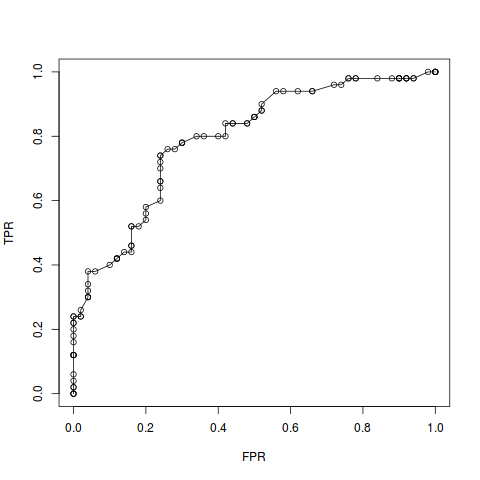The area under the ROC curve, or AUC, seems like a nice heuristic to evaluate and compare the overall performance of classification models independent of the exact decision threshold chosen. $$\mathrm{AUC} = 1.0$$ signifies perfect classification accuracy, and $$\mathrm{AUC} = 0.5$$ is the accuracy of making classification decisions via coin toss (or rather a continuous coin that outputs values in $$[0,1]$$…). Most classification algorithms will result in an AUC in that range. But there’s more to it.

## Probabilistic interpretation

As above, assume that we are looking at a dataset where we want to distinguish data points of type 0 from those of type 1. Consider a classification algorithm that assigns to a random observation $$\mathbf{x}\in\mathbb{R}^p$$ a score (or probability) $$\hat{p}(\mathbf{x}) \in [0,1]$$ signifying membership in class 1. If the final classification between class 1 and class 0 is determined by a decision threshold $$t\in[0, 1]$$, then the true positive rate (a.k.a. sensitivity or recall) can be written as a conditional probability

$T(t) := P[\hat{p}(\mathbf{x}) > t \,|\, \mathbf{x}\,\text{belongs to class 1}],$

and the false positive rate (or 1 - specificity) can be written as

$F(t) := P[\hat{p}(\mathbf{x}) > t \,|\, \mathbf{x}\,\text{does not belong to class 1}].$

For brevity of notation let’s say $$y(\mathbf{x}) = 1$$ instead of “$$\mathbf{x}$$ belongs to class 1”, and $$y(\mathbf{x})=0$$ instead of “$$\mathbf{x}$$ doesn’t belong to class 1”.

The ROC curve simply plots $$T(t)$$ against $$F(t)$$ while varying $$t$$ from 0 to 1. Thus, if we view $$T$$ as a function of $$F$$, the AUC can be rewritten as follows.

$\begin{eqnarray} \mathrm{AUC} &=& \int_0^1 T(F_0) \,\mathrm{d}F_0 \nonumber \\ &=& \int_0^1 P[\hat{p}(\mathbf{x}) > F^{-1}(F_0) \,|\, y(\mathbf{x}) = 1] \,\mathrm{d}F_0 \nonumber \\ &=& \int_1^0 P[\hat{p}(\mathbf{x}) > F^{-1}(F(t)) \,|\, y(\mathbf{x}) = 1] \cdot \frac{\partial F(t)}{\partial t} \,\mathrm{d}t \nonumber \\ &=& \int_0^1 P[\hat{p}(\mathbf{x}) > t \,|\, y(\mathbf{x}) = 1] \cdot P[\hat{p}(\mathbf{x^{\prime}}) = t \,|\, y(\mathbf{x^{\prime}}) = 0] \,\mathrm{d}t \nonumber \\ &=& \int_0^1 P[\hat{p}(\mathbf{x}) > \hat{p}(\mathbf{x^{\prime}}) \,\&\, \hat{p}(\mathbf{x^{\prime}}) = t \,|\, y(\mathbf{x}) = 1 \,\&\, y(\mathbf{x^{\prime}}) = 0] \,\mathrm{d}t \nonumber \\ &=& P[\hat{p}(\mathbf{x}) > \hat{p}(\mathbf{x^{\prime}}) \,|\, y(\mathbf{x}) = 1 \,\&\, y(\mathbf{x^{\prime}}) = 0], \nonumber \end{eqnarray}$

where we used the fact that the probability density function

$P[\hat{p}(\mathbf{x^{\prime}}) = t \,|\, y(\mathbf{x^{\prime}}) = 0] =: f(t)$

is the derivative with respect to $$t$$ of the cumulative distribution function

$P[\hat{p}(\mathbf{x^{\prime}}) \leq t \,|\, y(\mathbf{x^{\prime}}) = 0] = 1-F(t).$

So, given a randomly chosen observation $$\mathbf{x}$$ belonging to class 1, and a randomly chosen observation $$\mathbf{x^{\prime}}$$ belonging to class 0, the AUC is the probability that the evaluated classification algorithm will assign a higher score to $$\mathbf{x}$$ than to $$\mathbf{x^{\prime}}$$, i.e., the conditional probability of $$\hat{p}(\mathbf{x}) > \hat{p}(\mathbf{x^{\prime}})$$.

An alternative purely geometric proof can be found in the Scatterplot Smoothers blog.

In other words, if the classification algorithm distinguishes “positive” and “negative” examples (e.g., disease status), then

AUC is the probability of correct ranking of a random “positive”-“negative” pair.

## Computing AUC

The above probabilistic interpretation suggest a simple formula to compute AUC on a finite sample:

Among all “positive”-“negative” pairs in the dataset compute the proportion of those which are ranked correctly by the evaluated classification algorithm.

Here is an inefficient implementation using results from the above logistic regression example:

s <- 0
for (i in which(y == 1)) {
for (j in which(y == 0)) {
if (y_pred[i] > y_pred[j]) {
s <- s + 1
} else if (y_pred[i] == y_pred[j]) {
s <- s + 0.5
}
}
}
s <- s / (sum(y == 1) * sum(y == 0))
s
#  0.7918


The proportion of correctly ranked “positive”-“negative” pairs yields estimated $$\mathrm{AUC} = 0.7918$$.

We can compare this value to the area under the ROC curve computed with the trapezoidal rule.

s <- 0
for (i in 1:(length(FPR) - 1)) {
dFPR <- abs(FPR[i+1] - FPR[i])
s <- s + 0.5 * dFPR * (TPR[i+1] + TPR[i])
}
s
#  0.7922


Trapezoidal rule yields estimated $$\mathrm{AUC} = 0.7922$$. The difference of $$0.0004$$ can be explained by the fact that we evaluated the ROC curve at only 100 points.

Since there is a minor disagreement, let’s use some standard R package to compute AUC.

library(ROCR)
pred <- prediction(y_pred, y)
auc <- as.numeric(performance(pred, measure = "auc")@y.values)
auc
#  0.7918


Same as the proportion of correctly ranked pairs! :grin:

#### Wilcoxon-Mann-Whitney test

By analysing the probabilistic meaning of AUC, we not only got a practically relevant interpretation of this classification performance metric, but we also obtained a simple formula to estimate the AUC of a trained classification algorithm. Well, it turns out that taking the proportion of correctly ranked “positive”-“negative” pairs as a formula to estimate the AUC is equivalent to the Wilcoxon-Mann-Whitney statistical test. This fact can also be easily demonstrated in a couple lines of R code.

y_is_1 <- which(y == 1)
y_is_0 <- which(y == 0)
n_pairs <- length(y_is_1) * length(y_is_0)
WMW_test <- wilcox.test(y_pred[y_is_1], y_pred[y_is_0])
WMW_test$statistic / n_pairs # W # 0.7918  Same answer! ## So what? Why care about AUC anyway? • It has a really nice probabilistic meaning! :wink: Besides (arguably more importantly), as a measure of classification performance AUC has many advantages compared to other “single number” performance measures: • Independence of the decision threshold. • Invariance to prior class probabilities or class prevalence in the data. • Can choose/change a decision threshold based on cost-benefit analysis after model training. • Extensively used in machine learning, and in medical research – and that for good reasons, as for example explained in an excellent blog post on deep learning research in medicine by Luke Oakden-Rayner. ]]> Alexej Gossmann Mining USPTO full text patent data - An exploratory analysis of machine learning and AI related patents granted in 2017 so far2017-09-22T00:00:00-04:002017-09-22T00:00:00-04:00https://www.alexejgossmann.com/patents_part_1The United States Patent and Trademark office (USPTO) provides immense amounts of data (the data I used are in the form of XML files). After coming across these datasets, I thought that it would be a good idea to explore where and how my areas of interest fall into the intellectual property space; my areas of interest being machine learning (ML), data science, and artificial intelligence (AI). I started this exploration by downloading the full text data (excluding images) for all patents that were assigned by the USPTO within the year 2017 up to the time of writing (Patent Grant Full Text Data/XML for the year 2017 through the week of Sept 12 from the USPTO Bulk Data Storage System). In this blog post I address questions such as: How many ML and AI related patents were granted? Who are the most prolific inventors? The most frequent patent assignees? Where are inventions made? And when? Is the number of ML and AI related patents increasing over time? How long does it take to obtain a patent for a ML or AI related invention? Is the patent examination time shorter for big tech companies? Etc. First, I curated a patent full text dataset consisting of “machine learning and AI related” patents. I am not just looking for instances where actual machine learning or AI algorithms were patented; I am looking for inventions which are related to ML or AI in any/some capacity. That is, I am interested in patents where machine learning, data mining, predictive modeling, or AI is utilized as a part of the invention in any way whatsoever. The subset of relevant patents was determined by a keyword search as specified by the following definition. Definition: For the purposes of this blog post, a machine learning or AI related patent is a patent that contains at least one of the keywords “machine learning”, “deep learning”, “neural network”, “artificial intelligence”, “statistical learning”, “data mining”, or “predictive model” in its invention title, description, or claims text (while of course accounting for capitalization, pluralization, etc.).1 With this keyword matching approach a total of 6665 patents have been selected. The bar graph below shows how many times each keyword got matched.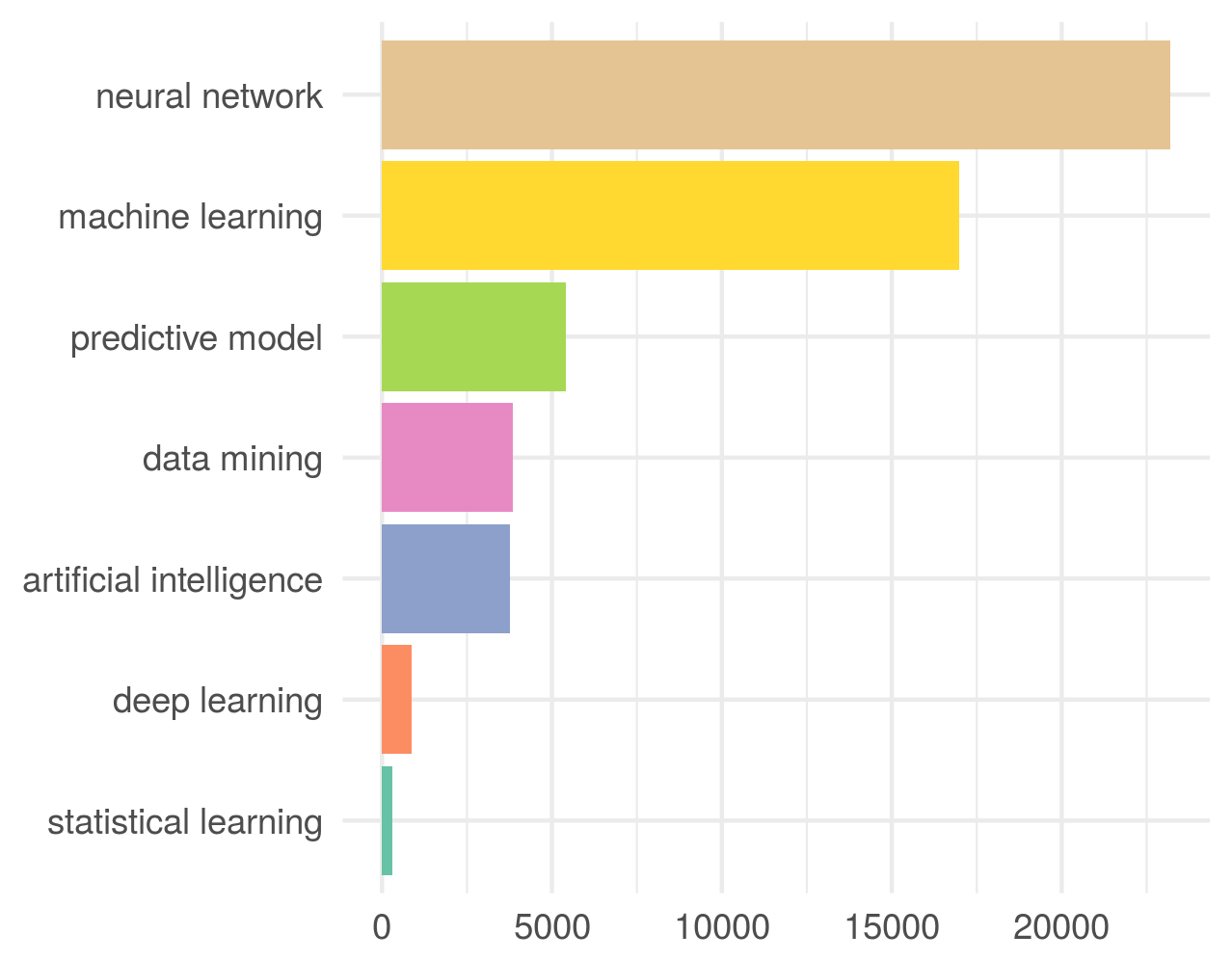Interestingly the term “neural network” is even more common than the more general terms “machine learning” and “artificial intelligence”. ### Some example patents Here are three (randomly chosen) patents from the resulting dataset. For each printed are the invention title, the patent assignee, as well as one instance of the keyword match within the patent text. And here are three examples of (randomly picked) patents that contain the relevant keywords directly in their invention title. ## Who holds these patents (inventors and assignees)? The first question I would like to address is who files most of the machine learning and AI related patents. Each patent specifies one or several inventors, i.e., the individuals who made the patented invention, and a patent assignee which is typically the inventors’ employer company that holds the rights to the patent. The following bar graph visualizes the top 20 most prolific inventors and the top 20 most frequent patent assignees among the analyzed ML and AI related patents.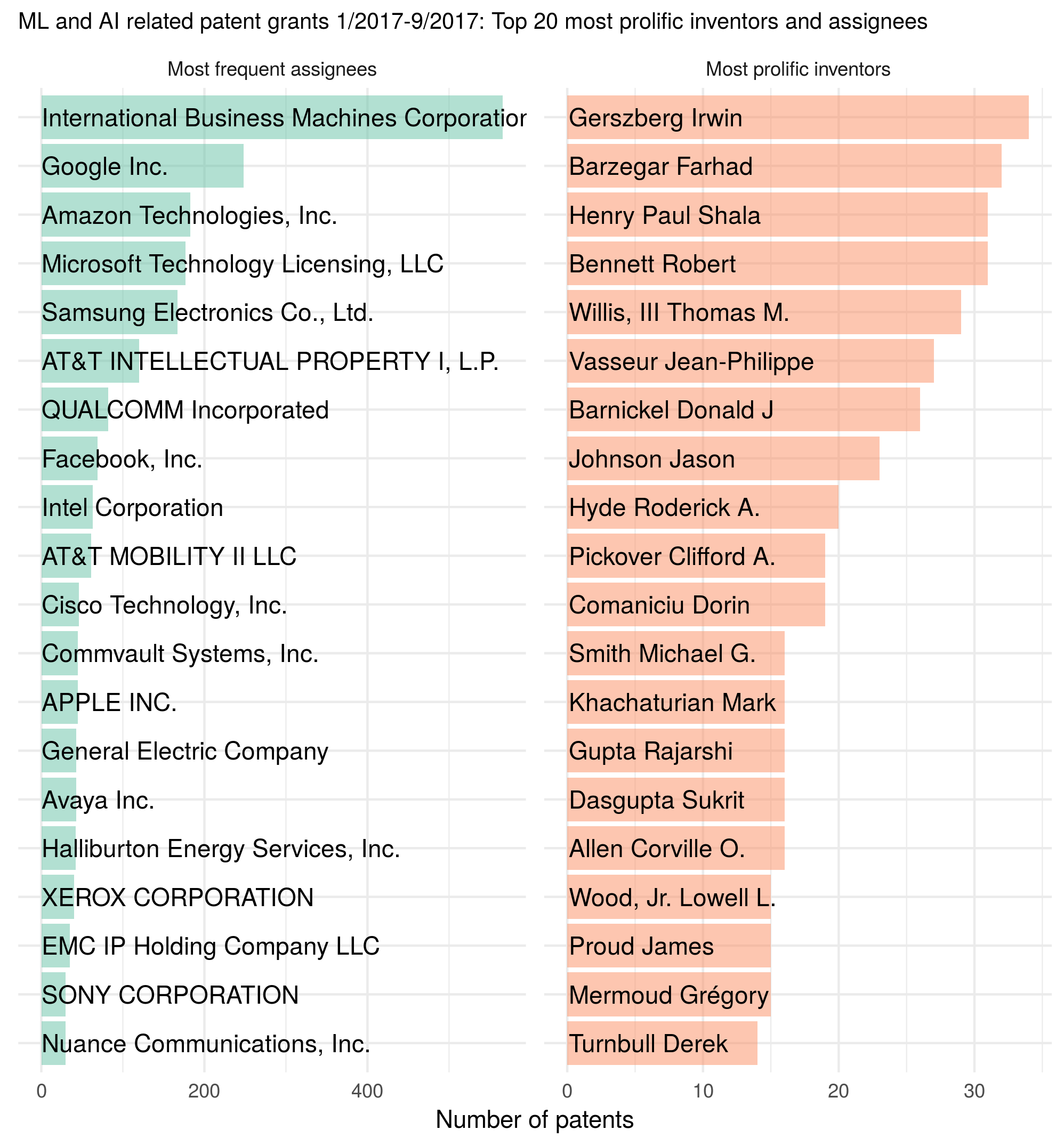It isn’t surprising to see this list of companies. The likes of IBM, Google, Amazon, Microsoft, Samsung, and AT&T rule the machine learning and AI patent space. I have to admit that I don’t recognize any of the inventors’ names (but it might just be me not being familiar enough with the ML and AI community). There are a number of interesting follow-up questions which for now I leave unanswered (hard to answer without additional data): • What is the count of ML and AI related patents by industry or type of company (e.g., big tech companies vs. startups vs. reserach universities vs. government)? • Who is deriving the most financial benefit by holding ML or AI related patents (either through licensing or by driving out the competition)? ## Where do these inventions come from (geographically)? Even though the examined patents were filed in the US, some of the inventions may have been made outside of the US. In fact, the data includes specific geographic locations for each patent, so I can map in which cities within the US and the world inventors are most active. The following figure is based on where the inventors are from, and shows the most active spots. Each point corresponds to the total number of inventions made at that location (though note that the color axis is a log10 scale, and so is the point size).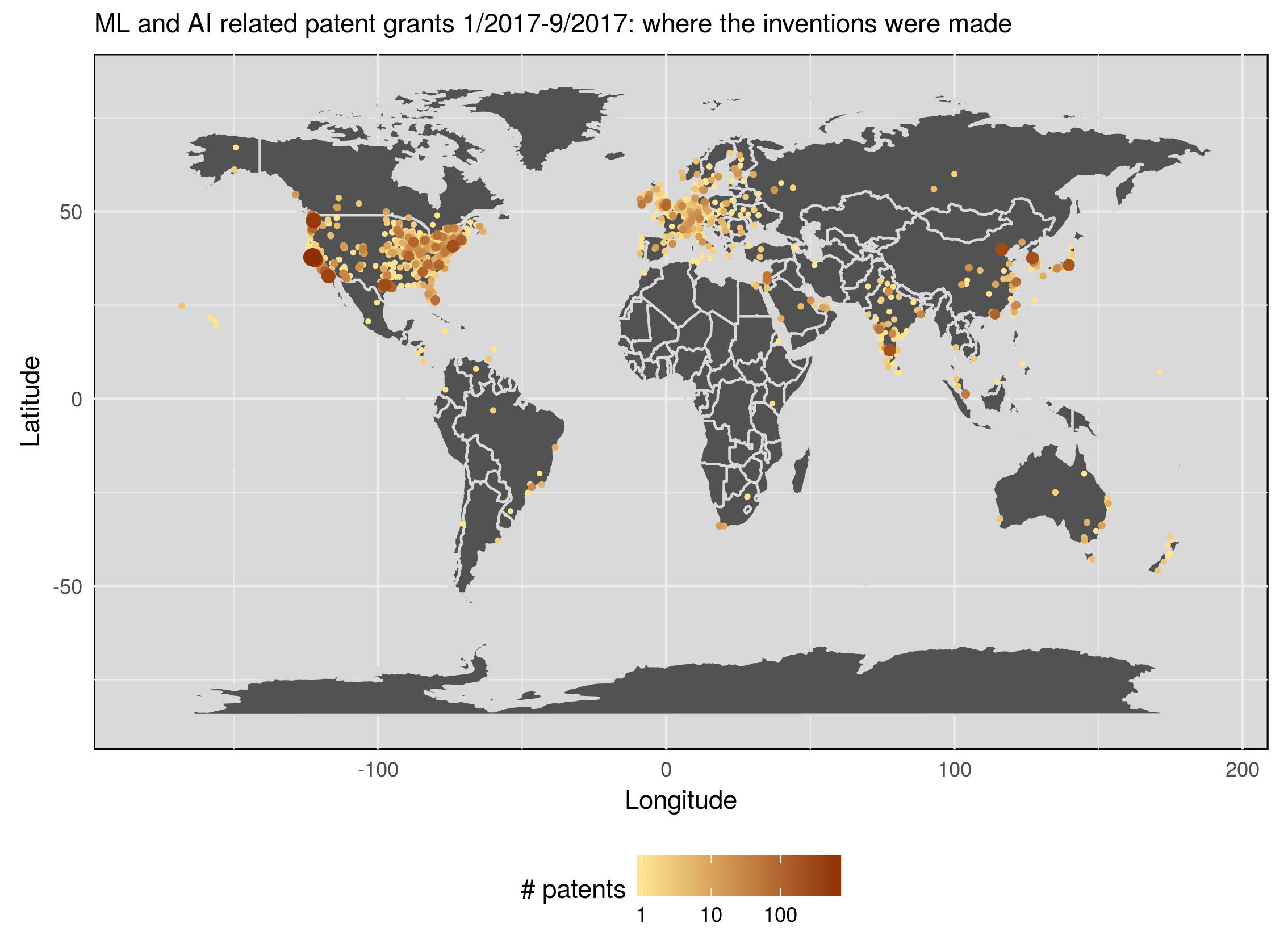The results aren’t that surprising. However, we see that most (ML and AI related) inventions patented with the USPTO were made in the US. I wonder if inventors in other countries prefer to file patents in their home countries’ patent offices rather the in the US. Alternatively, we can also map the number of patents per inventors’ origin countries.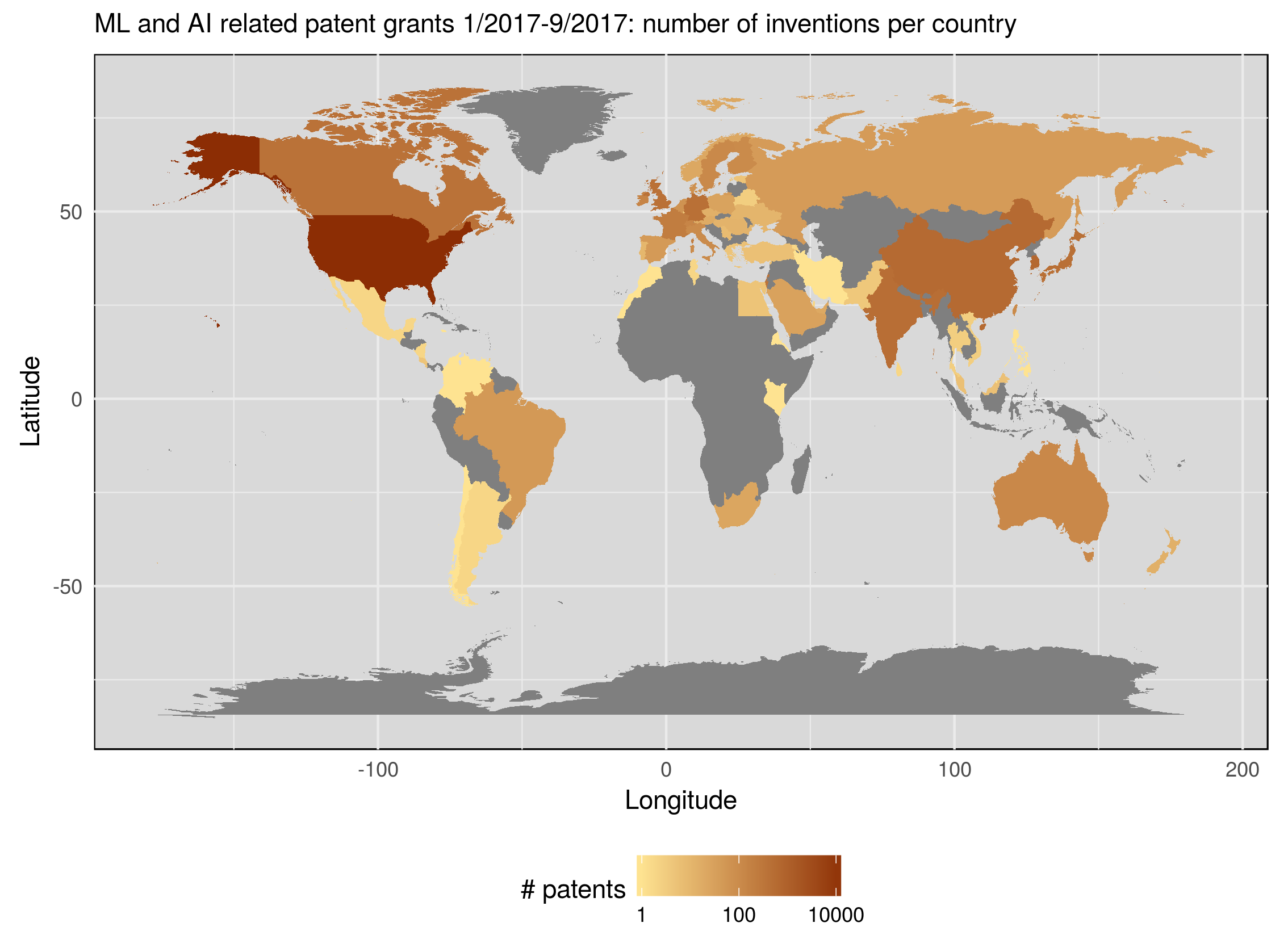Sadly, there seem to be entire groups of countries (e.g., almost the entire African continent) which seem to be excluded from the USPTO’s patent game, at least with respect to machine learning and AI related inventions. Whether it is a lack of access, infrastructure, education, political treaties or something else is an intriguing question. ## Patent application and publication dates, and duration of patent examination process Each patent has a date of filing and an assignment date attached to it. Based on the provided dates one can try to address questions such as: When were these patents filed? Is the number of ML and AI related patents increasing over time? How long did it usually take from patent filing to assignment? And so on. For the set of ML and AI related patents that were granted between Jan 3 and Sept 12 2017 the following figure depicts… • …in the top panel: number of patents (y-axis) per their original month of filing (x-axis), • …in the bottom panel: the number of patents (y-axis) that were assigned (approved) per week (x-axis) in 2017 so far.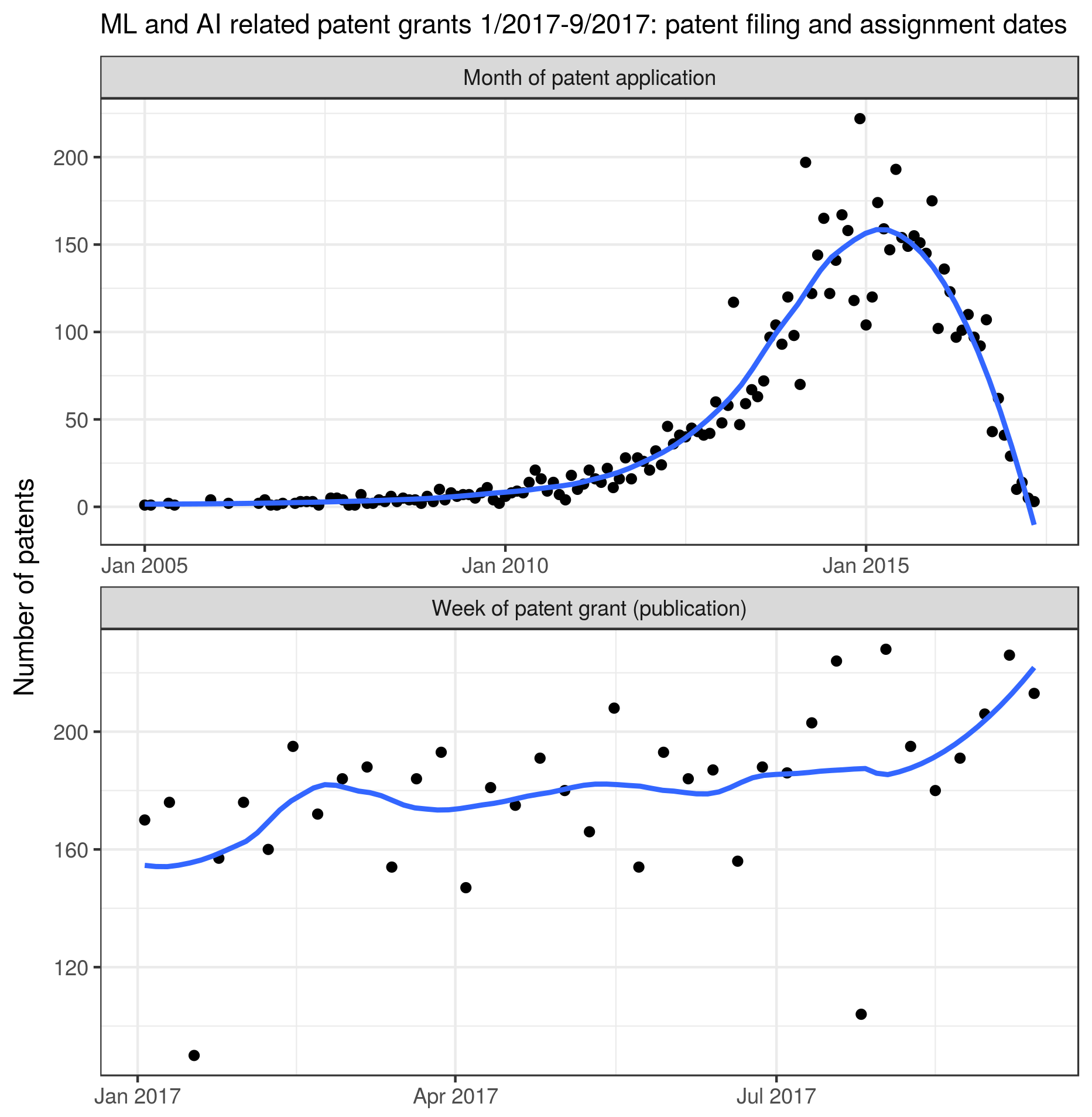The patent publication dates plot suggests that the number of ML and AI related patents seems to be increasing slightly throughout the year 2017. The patent application dates plot suggests that the patent examination phase for the considered patents takes about 2.5 years. In fact the average time from patent filing to approval is 2.83 years with a standard deviation of 1.72 years in this dataset (that is, among the considered ML and AI related patents in 2017). However, the range is quite extensive spanning 0.24-12.57 years. The distribution of the duration from patent filing date to approval is depicted by following figure.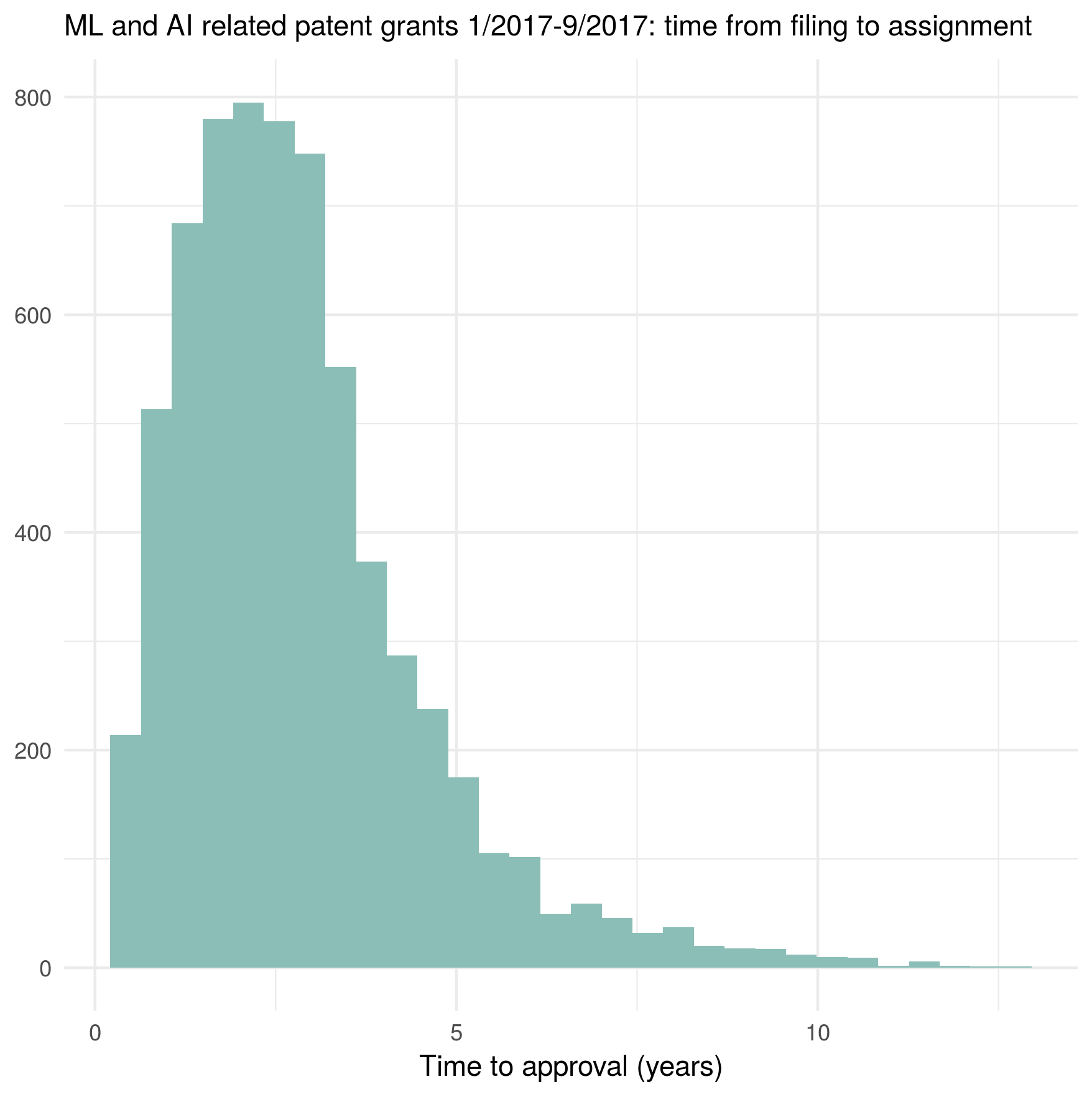So, what are some of the inventions that took longest to get approved? Here are the five patents with the longest examination periods: • “Printing and dispensing system for an electronic gaming device that provides an undisplayed outcome” (~12.57 years to approval; assignee: Aim Management, Inc.) • “Apparatus for biological sensing and alerting of pharmaco-genomic mutation” (~12.24 years to approval; assignee: NA) • “System for tracking a player of gaming devices” (~12.06 years to approval; assignee: Aim Management, Inc.) • “Device, method, and computer program product for customizing game functionality using images” (~11.72 years to approval; assignee: NOKIA TECHNOLOGIES OY) • “Method for the spectral identification of microorganisms” (~11.57 years to approval; assignee: MCGILL UNIVERSITY, and HEALTH CANADA) Each of these patents is related to either gaming or biotech. I wonder if that’s a coincidence… We can also look at the five patents with the shortest approval time: • “Home device application programming interface” (~91 days to approval; assignee: ESSENTIAL PRODUCTS, INC.) • “Avoiding dazzle from lights affixed to an intraoral mirror, and applications thereof” (~104 days to approval; assignee: DENTAL SMARTMIRROR, INC.) • “Media processing methods and arrangements” (~106 days to approval; assignee: Digimarc Corporation) • “Machine learning classifier that compares price risk score, supplier risk score, and item risk score to a threshold” (~111 days to approval; assignee: ACCENTURE GLOBAL SOLUTIONS LIMITED) • “Generating accurate reason codes with complex non-linear modeling and neural networks” (~111 days to approval; assignee: SAS INSTITUTE INC.) Interstingly the patent approved in the shortest amount of time among all 6665 analysed (ML and AI related) patents is some smart home thingy from Andy Rubin’s hyped up company Essential. ### Do big tech companies get their patents approved faster than other companies (e.g., startups)? The following figure separates the patent approval times according to the respective assignee company, considering several of the most well known tech giants.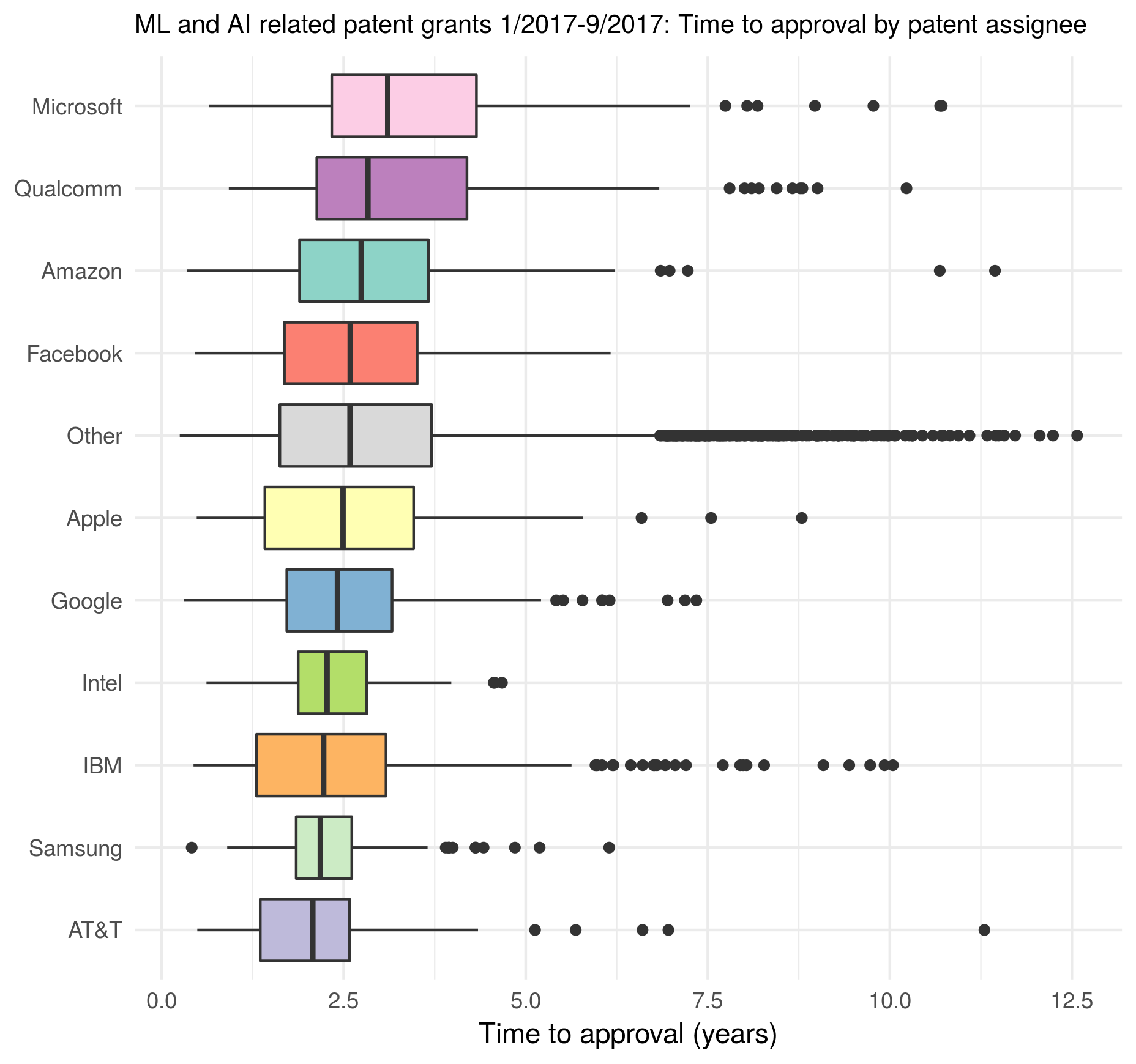Indeed some big tech companies, such as AT&T or Samsung, manage to push their patent application though the USPTO process much faster than most other companies. However, there are other tech giants, such as Microsoft, which on average take longer to get their patent applications approved than even the companies in category “Other”. Also noteworthy is the fact that big tech companies tend to have fewer outliers regarding the patent examination process duration than companies in the category “Other”. Of course it would also be interesting to categorize all patent assignees into categories like “Startup”, “Big Tech”, “University”, or “Government”, and compare the typical duration of the patent examination process between such groups. However, it’s not clear to me how to establish such categories without collecting additional data on each patent assignee, which at this point I don’t have time for :stuck_out_tongue:. ## Conclusion There is definitely a lot of promise in the USPTO full text patent data. Here I have barely scratched the surface, and I hope that I will find the time to play around with these data some more. The end goal is, of course, to replace the patent examiner with an AI trained on historical patent data. :stuck_out_tongue_closed_eyes:This work (blog post and included figures) is licensed under a Creative Commons Attribution 4.0 International License. 1. There are two main aspects to my reasoning as to this particular choice of keywords. (1) I wanted to keep the list relatively short in order to have a more specific search, and (2) I tried to avoid keywords which may generate false positives (e.g., the term “AI” would match all sorts of codes present in the patent text, such as “123456789 AI N1”). In no way am I claiming that this is a perfect list of keywords to identify ML and AI related patents, but I think that it’s definitely a good start. ]]> Alexej Gossmann Freedman’s paradox2017-06-05T20:00:00-04:002017-06-05T20:00:00-04:00https://www.alexejgossmann.com/Freedmans_paradoxRecently I came across the classical 1983 paper A note on screening regression equations by David Freedman. Freedman shows in an impressive way the dangers of data reuse in statistical analyses. The potentially dangerous scenarios include those where the results of one statistical procedure performed on the data are fed into another procedure performed on the same data. As a concrete example Freedman considers the practice of performing variable selection first, and then fitting another model using only the identified variables on the same data that was used to identify them in the first place. Because of the unexpectedly high severity of the problem this phenomenon became known as “Freedman’s paradox”. Moreover, in his paper Freedman derives asymptotic estimates for the resulting errors. The 1983 paper presents a simulation with only 10 repetitions. But in the present day it is very easy (both in terms of computational time and implementation difficulty) to reproduce the simulation with many more repetitions (even my phone’s computational power is probably higher than that of the high performance computer that Freedman used in the 80’s). We also have more convenient ways to visualize the results than in the 80’s. So let’s do it. I am going to use a few R packages (most notably the package broom to fit and analyze many many linear models in a single step). library(dplyr) library(broom) library(ggplot2) library(tidyr) set.seed(20170605)  The considered data structure is the following: • A matrix of predictors with 100 rows and 50 columns is generated with independent standard normal entries. • The response variable is generated independently of the model matrix (also from the standard normal distribution), i.e., the true answer is that there is no relationship between predictors and response. Instead of Freedman’s 10 repetitions we perform 1000. So let’s generate all 1000 datasets at once as stacked in a large data frame: n_row <- 100 # n_col is set to 51 because the 51st column will serve as y n_col <- 51 n_rep <- 1000 # a stack of matrices for all n_rep repetitions is generated... X <- matrix(rnorm(n_rep * n_row * n_col), n_rep * n_row, n_col) colnames(X) <- paste0("X", 1:n_col) # ...and then transformed to a data frame with a repetition number column X_df <- as_data_frame(X) %>% mutate(repetition = rep(1:n_rep, each = n_row))  The data are analyzed in two successive linear models. The second (illegally) reusing the results of the first. The first model fit. After the 1000 ordinary linear models are fit to the data, we record for each of them the R squared, the F test statistic with corresponding p-value, and the t test statistics with p-values for the individual regression coefficients. Using functions from the broom package we can fit and extract information from all 1000 models at once. # all models can be fit at once... models_df = X_df %>% group_by(repetition) %>% do(full_model = lm(X51 ~ . + 0, data = select(., -repetition))) # ...then the results are extracted model_coefs <- tidy(models_df, full_model) model_statistics <- glance(models_df, full_model) model_statistics$data_reuse <- rep(FALSE, nrow(model_statistics))


The second model fit. For each one of the first 1000 models, the corresponding second linear model is fit using only those variables which have p-values significant at the 25% level in the first model. That is, the second model uses the first model for variable selection.

This gives us 1000 reduced re-fitted linear models. We record the same model statistics (R squared, F, and t tests) as for the first group of models.

reduced_models <- list()
for (i in 1:n_rep) {
full_data <- X_df %>% filter(repetition == i)
significant_coefs <- model_coefs %>%
filter(repetition == i & p.value < 0.25)
reduced_data <- select(full_data,
one_of(unlist(significant_coefs[ , "term"])), X51)
reduced_models[[i]] <- lm(X51 ~ . + 0, data = reduced_data)
tmp_df <- glance(reduced_models[[i]])
tmp_df$repetition <- i tmp_df$data_reuse <- TRUE
model_statistics <- bind_rows(model_statistics, tmp_df)
}


Finally let’s look at the results. The figure shows the distributions of the considered model statistics across the 1000 repetitions for model fits with and without data reuse (the code producing this figure is given at the bottom of this post):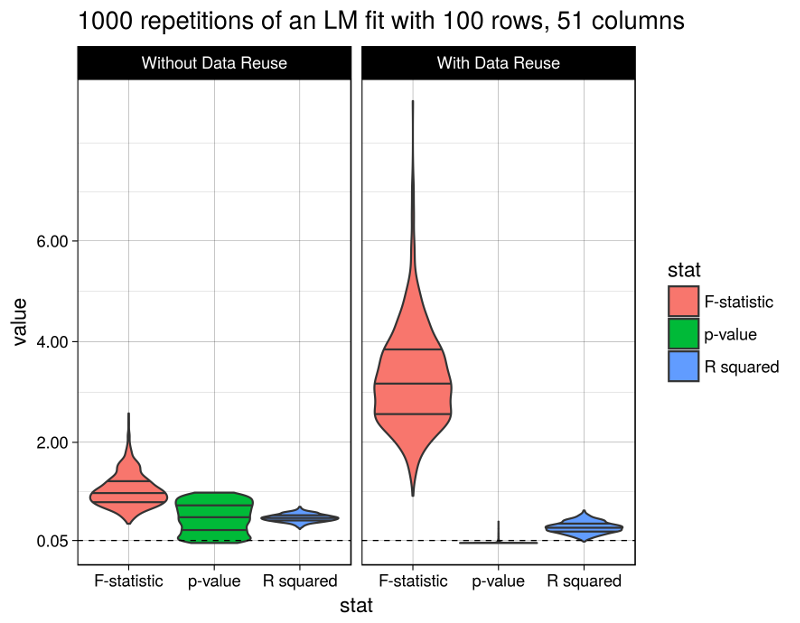Well, the R squared statistic shows a moderate change between models with or without data reuse (average of 0.3093018 vs. 0.5001641). The F test statistic however grows immensely to an average of 3.2806118 (from 1.0480097), and the p-values fall after data reuse to an average of 0.0112216 (from 0.5017696), below the widely used (but arbitrary) 5% significance level.

Obviously the model with data reuse is highly misleading here, because in fact there are absolutely no relationships between the predictor variables and the response (as per the data generation procedure).

In fact, Freedman derived asymptotic estimates for the magnitudes of change in the considered model statistics, and they indeed match the above observations. However I’m too lazy to summarize them here. So I refer to the primary source.

This code generates the above figure:

model_statistics %>%
select(r.squared, p.value, statistic, repetition, data_reuse) %>%
mutate(data_reuse = ifelse(data_reuse, "With Data Reuse", "Without Data Reuse")) %>%
mutate(data_reuse = factor(data_reuse, levels = c("Without Data Reuse", "With Data Reuse"),
ordered = TRUE)) %>%
rename("F-statistic" = statistic, "p-value" = p.value, "R squared" = r.squared) %>%
gather(stat, value, -repetition, -data_reuse) %>%
ggplot(aes(x = stat, y = value)) +
geom_violin(aes(fill = stat), scale = "width", draw_quantiles = c(0.25, 0.5, 0.75)) +
geom_hline(yintercept = 0.05, linetype = 2, size = 0.3) +
facet_wrap(~data_reuse) +
theme_linedraw() +
scale_y_continuous(breaks = c(0.05, 2, 4, 6)) +
ggtitle(paste(n_rep, "repetitions of an LM fit with", n_row, "rows,", n_col, "columns"))

]]>
Alexej Gossmann
5 ways to measure running time of R code2017-05-28T00:09:00-04:002017-05-28T00:09:00-04:00https://www.alexejgossmann.com/benchmarking_rA reviewer asked me to report detailed running times for all (so many :scream:) performed computations in one of my papers, and so I spent a Saturday morning figuring out my favorite way to benchmark R code. This is a quick summary of the options I found to be available.

A quick online search revealed at least three R packages for benchmarking R code (rbenchmark, microbenchmark, and tictoc). Additionally, base R provides at least two methods to measure the running time of R code (Sys.time and system.time). In the following I briefly go through the syntax of using each of the five option, and present my conclusions at the end.

### 1. Using “Sys.time”

The run time of a chunk of code can be measured by taking the difference between the time at the start and at the end of the code chunk. Simple yet flexible :sunglasses:.

sleep_for_a_minute <- function() { Sys.sleep(60) }

start_time <- Sys.time()
sleep_for_a_minute()
end_time <- Sys.time()

end_time - start_time
# Time difference of 1.000327 mins


### 2. Library “tictoc”

The functions tic and toc are used in the same manner for benchmarking as the just demonstrated Sys.time. However tictoc adds a lot more convenience to the whole.

The most recent development version of tictoc can be installed from github:

devtools::install_github("jabiru/tictoc")


One can time a single code chunk:

library(tictoc)

tic("sleeping")
print("falling asleep...")
sleep_for_a_minute()
print("...waking up")
toc()
#  "falling asleep..."
#  "...waking up"
# sleeping: 60.026 sec elapsed


Or nest multiple timers:

tic("total")
tic("data generation")
X <- matrix(rnorm(50000*1000), 50000, 1000)
b <- sample(1:1000, 1000)
y <- runif(1) + X %*% b + rnorm(50000)
toc()
tic("model fitting")
model <- lm(y ~ X)
toc()
toc()
# data generation: 3.792 sec elapsed
# model fitting: 39.278 sec elapsed
# total: 43.071 sec elapsed


### 3. Using “system.time”

One can time the evaluation of an R expression using system.time. For example, we can use it to measure the execution time of the function sleep_for_a_minute (defined above) as follows.

system.time({ sleep_for_a_minute() })
#   user  system elapsed
#  0.004   0.000  60.051


But what exactly are the reported times user, system, and elapsed? :confused:

Well, clearly elapsed is the wall clock time taken to execute the function sleep_for_a_minute, plus some benchmarking code wrapping it (that’s why it took slightly more than a minute to run I guess).

As for user and system times, William Dunlap has posted a great explanation to the r-help mailing list:

“User CPU time” gives the CPU time spent by the current process (i.e., the current R session) and “system CPU time” gives the CPU time spent by the kernel (the operating system) on behalf of the current process. The operating system is used for things like opening files, doing input or output, starting other processes, and looking at the system clock: operations that involve resources that many processes must share. Different operating systems will have different things done by the operating system.

:grinning:

### 4. Library “rbenchmark”

The documentation to the function benchmark from the rbenchmark R package describes it as “a simple wrapper around system.time”. However it adds a lot of convenience compared to bare system.time calls. For example it requires just one benchmark call to time multiple replications of multiple expressions. Additionally the returned results are conveniently organized in a data frame.

I installed the development[^1] version of the rbenchmark package from github:

devtools::install_github("eddelbuettel/rbenchmark")


For example purposes, let’s compare the time required to compute linear regression coefficients using three alternative computational procedures:

1. lm,
2. the Moore-Penrose pseudoinverse,
3. the Moore-Penrose pseudoinverse but without explicit matrix inverses.
library(rbenchmark)

benchmark("lm" = {
X <- matrix(rnorm(1000), 100, 10)
y <- X %*% sample(1:10, 10) + rnorm(100)
b <- lm(y ~ X + 0)$coef }, "pseudoinverse" = { X <- matrix(rnorm(1000), 100, 10) y <- X %*% sample(1:10, 10) + rnorm(100) b <- solve(t(X) %*% X) %*% t(X) %*% y }, "linear system" = { X <- matrix(rnorm(1000), 100, 10) y <- X %*% sample(1:10, 10) + rnorm(100) b <- solve(t(X) %*% X, t(X) %*% y) }, replications = 1000, columns = c("test", "replications", "elapsed", "relative", "user.self", "sys.self")) # test replications elapsed relative user.self sys.self # 3 linear system 1000 0.167 1.000 0.208 0.240 # 1 lm 1000 0.930 5.569 0.952 0.212 # 2 pseudoinverse 1000 0.240 1.437 0.332 0.612  Here, the meaning of elapsed, user.self, and sys.self is the same as described above in the section about system.time, and relative is simply the time ratio with the fastest test. Interestingly lm is by far the slowest here. ### 5. Library “microbenchmark” The most recent development version of microbenchmark can be installed from github: devtools::install_github("olafmersmann/microbenchmarkCore") devtools::install_github("olafmersmann/microbenchmark")  Much like benchmark from the package rbenchmark, the function microbenchmark can be used to compare running times of multiple R code chunks. But it offers a great deal of convenience and additional functionality. I find that one particularly nice feature of microbenchmark is the ability to automatically check the results of the benchmarked expressions with a user-specified function. This is demonstrated below, where we again compare three methods computing the coefficient vector of a linear model. library(microbenchmark) set.seed(2017) n <- 10000 p <- 100 X <- matrix(rnorm(n*p), n, p) y <- X %*% rnorm(p) + rnorm(100) check_for_equal_coefs <- function(values) { tol <- 1e-12 max_error <- max(c(abs(values[] - values[]), abs(values[] - values[]), abs(values[] - values[]))) max_error < tol } mbm <- microbenchmark("lm" = { b <- lm(y ~ X + 0)$coef },
"pseudoinverse" = {
b <- solve(t(X) %*% X) %*% t(X) %*% y
},
"linear system" = {
b <- solve(t(X) %*% X, t(X) %*% y)
},
check = check_for_equal_coefs)

mbm
# Unit: milliseconds
#           expr      min        lq      mean    median        uq      max neval cld
#             lm 96.12717 124.43298 150.72674 135.12729 188.32154 236.4910   100   c
#  pseudoinverse 26.61816  28.81151  53.32246  30.69587  80.61303 145.0489   100  b
#  linear system 16.70331  18.58778  35.14599  19.48467  22.69537 138.6660   100 a


We used the function argument check to check for equality (up to a maximal error of 1e-12) of the results returned by the three methods. If the results weren’t equal, microbenchmark would return an error message.

Another great feature is the integration with ggplot2 for plotting microbenchmark results.

library(ggplot2)
autoplot(mbm)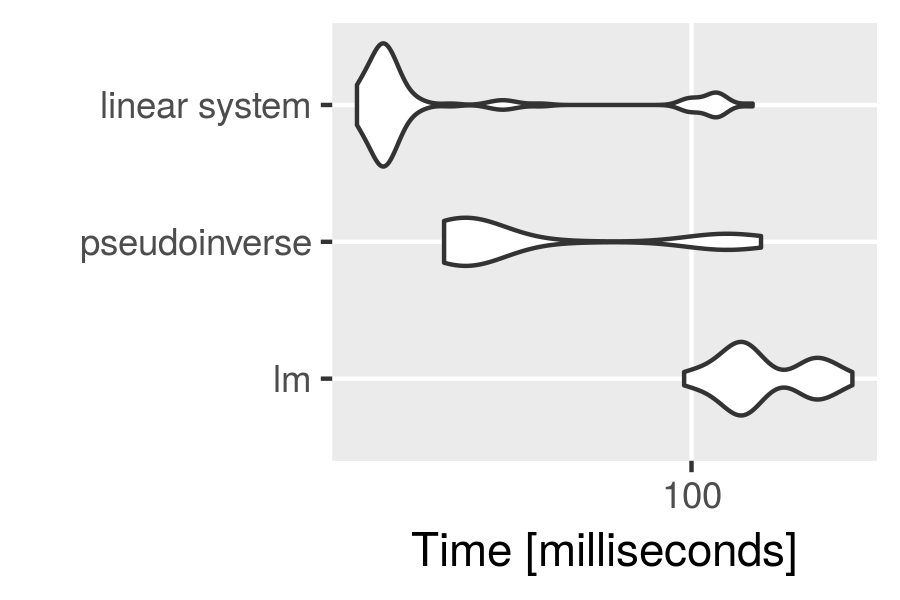### Conclusion

The given demonstration of the different benchmarking functions is surely not exhaustive. Nevertheless I made some conclusions for my personal benchmarking needs:

• The Sys.time approach as well as the tictoc package can be used for timing (potentially nested) steps of a complicated algorithm (that’s often my use case). However, tictoc is more convenient, and (most importantly) foolproof.
• We saw that microbenchmark returns other types of measurements than benchmark, and I think that in most situations the microbenchmark measurements are of a higher practical significance :stuck_out_tongue:.
• To my knowledge microbenchmark is the only benchmarking package that has visualizations built in :+1:.

For these reasons I will go with microbenchmark and tictoc. :bowtie:

]]>
Alexej Gossmann
The Lean PhD Student - Can The Lean Startup principles be applied to personal productivity in graduate school?2017-05-09T21:40:00-04:002017-05-09T21:40:00-04:00https://www.alexejgossmann.com/the-lean-phd-studentThe lean startup methodology consists of a set of principles that were proposed and popularized by Eric Ries in the book The Lean Startup (and elsewhere). He believes that startup success can be engineered by following the lean startup methodology. Eric Ries defines a startup as “a human institution designed to deliver a new product or service under conditions of extreme uncertainty”. If we replace “product or service” by “research result”, that sounds awfully similar to what a PhD student has to do. Indeed, the similarities between being a junior researcher, such as a PhD student, and running a startup have been often pointed out (for example: , , ). In light of this, I propose that the lean startup methodology can also be applied to academic pursuits of a PhD student. Below, I adapt some of the most important lean startup concepts for application to a junior researcher’s personal productivity and academic success.1

If we want to carry over startup concepts to academic research, then the first (and most obvious) question is, what would be the “product” and who would be the “customer” of the PhD student? I think the analogy here is quite straight forward. The “products” of a PhD student clearly are the student’s peer-reviewed publications, conference presentations, the dissertation, software releases, etc.; and the “customers” are other researchers and to a much smaller extent the general public. An especially important set of (quite often tough) “customers” includes the journal or conference paper reviewers and editors, and the student’s committee members.

### Build - Measure - Learn

At the center of the lean startup methodology is the so-called build-measure-learn feedback loop. One of the main goals of the lean startup methodology is to minimize the time (and other resources) required to pass through the build-measure-learn feedback loop, and to maximize the number of times that the build-measure-learn loop is completed. Its adaptation to academic research would be something like the following.

#### 1. Build

:hammer: Start with a novel idea, whose good execution you assume to be valuable to your scientific audience, and then share a minimally viable execution of the idea with members of your audience.

The concept of a minimum viable product (or MVP) is especially important during this stage of the lean startup trajectory, in order to minimize time spent in this stage. The Lean Startup defines the MVP as the “version of a new product which allows a team to collect the maximum amount of validated learning about customers with the least effort”. Analogously, I think that a minimum viable research result can for example consist of an exploration of the main idea on small samples, toy problems, and special cases, designed in such a way that would allow the researcher to obtain sufficient amount of feedback on his/her idea with the least effort.

#### 2. Measure

:triangular_ruler: Observe how other researchers react to your idea and its minimally viable execution.

In this step it is important to use so-called actionable metrics, as opposed to vanity metrics. Actionable metrics accurately reflect the key success factors of the project, while vanity metrics are measurements that give “the rosiest picture possible”. With regard to academic research actionable metrics may be (not an exhaustive list):

• Direct feedback from researchers who you trust.
• Others applying your work to their own research.
• Major contributions to high-quality peer-reviewed publications.

And (academic) vanity metrics may include:

• Co-authorships on other people’s papers, while having only slightly more contribution than none.
• Association or acquaintance with a “big name” scientist.
• Number of views of a researcher’s homepage, or paper view count on some online platform.
• Appearance in mainstream media.

Measuring the right metrics is a big part of what Eric Ries calls innovation accounting.

#### 3. Learn

:bulb: Learn how valuable your audience actually considers your idea to be based on the received feedback and your actionable metrics of choice. Utilize that new knowledge to improve the initial idea in order to make it more valuable to a targeted scientific audience, and adjust your assumptions about what your audience needs.

In The Lean Startup this type of a modification process on your initial idea is called a pivot, or in Eric Ries’ words: “A pivot is a structured course correction designed to test a new fundamental hypothesis about the product, strategy, and engine of growth.” Having obtained the corrected research idea, you would go back to step 1 and reiterate the whole process.

### Conclusion

So, what do we get out of all of this? I think that a clear strategy emerges here.

The strategy consists in striving to push results out fast, in order to receive feedback fast, which (feedback) is evaluated according to a suitable set of actionable metrics that were chosen in advance. That is, one needs to be writing papers fast, initially without worrying about things outside the scope of an MVP, such as the perfect word choice, the optimal formatting, coverage of all corner cases, etc., in order to get and measure the feedback from members of the target scientific audience quickly. Then the ideas need to be improved upon according to what was learned, and the process is reiterated. One of the major goals of the PhD student should be to minimize the time required to pass through this loop.

So is this a good strategy for a PhD student? Well, I can’t say before I try it out :stuck_out_tongue:. One crucial factor not mentioned here though is the PhD advisor. In my case I have a lot of freedom to come up with my own projects and pursue my own ideas as long as they are within a specific (but somewhat loosely-defined) area, and I could totally incorporate this lean startup inspired research strategy into my work. On the other extreme, there are professors who micromanage their PhD student’s every step, in which case the PhD student will find it much harder to experiment with their research strategy.

1. Please note that I’m writing from the point of view of mathematical, statistical, and computational sciences, rather than from the viewpoint of experimental sciences.

]]>
Alexej Gossmann
Salaries by alma mater - an interactive visualization with R and plotly2017-04-28T00:08:00-04:002017-04-28T00:08:00-04:00https://www.alexejgossmann.com/salaries_by_school_plotly_viz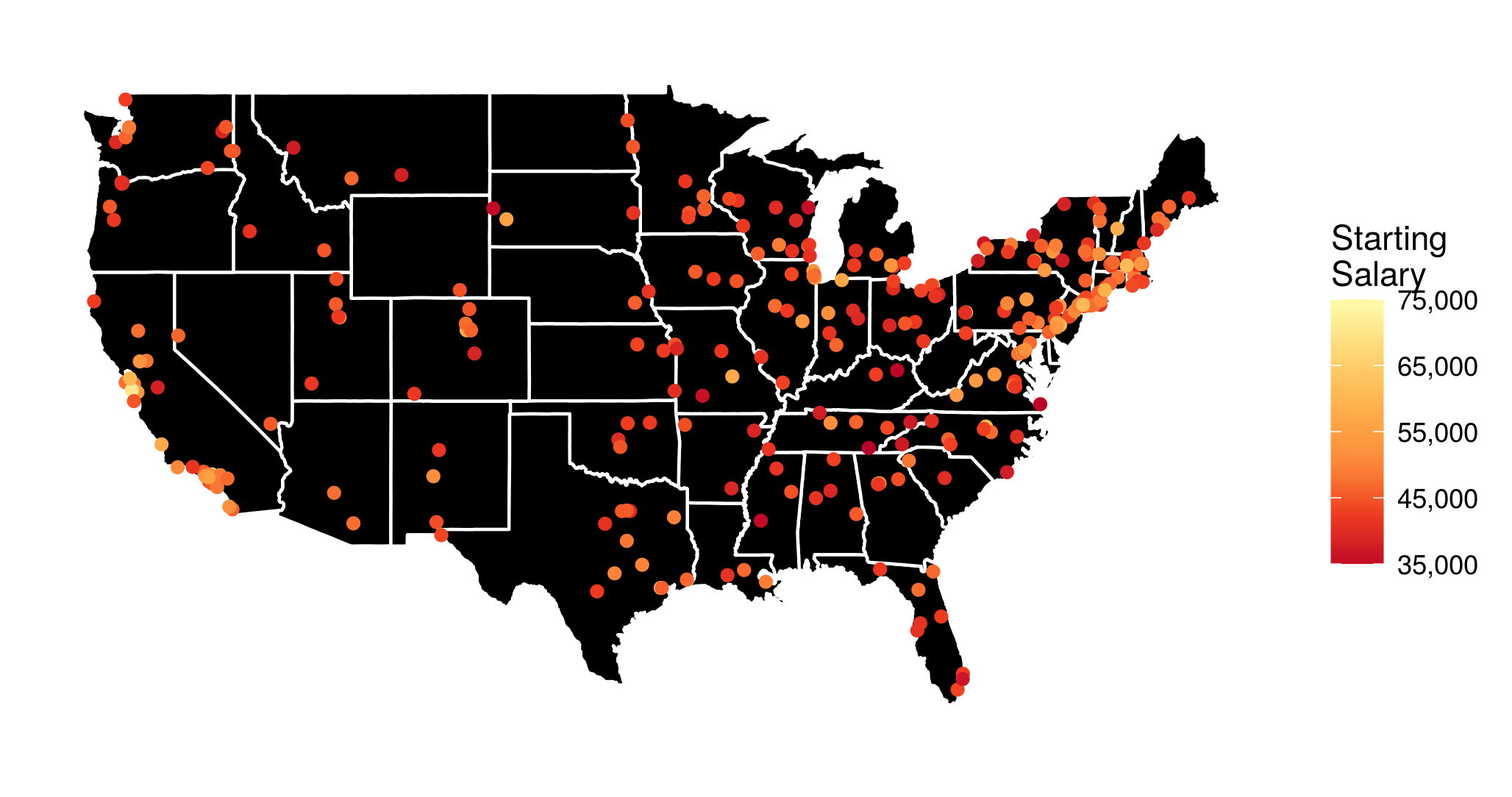Based on an interesting dataset from the Wall Street Journal I made the above visualization of the median starting salary for US college graduates from different undergraduate institutions (I have also looked at the mid-career salaries, and the salary increase, but more on that later). However, I thought that it would be a lot more informative, if it were interactive. To the very least I wanted to be able to see the school names when hovering over or clicking on the points with the mouse.

Luckily, this kind of interactivity can be easily achieved in R with the library plotly, especially due to its excellent integration with ggplot2, which I used to produce the above figure. In the following I describe how exactly this can be done.

Before I show you the interactive visualizations, a few words on the data preprocessing, and on how the map and the points are plotted with ggplot2:

• I generally use functions from the tidyverse R packages.
• I save the data in the data frame salaries, and transform the given amounts to proper floating point numbers, stripping the dollar signs and extra whitespaces.
• The data provide school names. However, I need to find out the exact geographical coordinates of each school to put it on the map. This can be done in a very convenient way, by using the geocode function from the ggmap R package:
school_longlat <- geocode(salaries$school) school_longlat$school <- salaries$school salaries <- left_join(salaries, school_longlat)  • For the visualization I want to disregard the colleges in Alaska and Hawaii to avoid shrinking the rest of the map. The respective rows of salaries can be easily determined with a grep search: grep("alaska", salaries$school, ignore.case = 1)
#  206
grep("hawaii", salaries\$school, ignore.case = 1)
#  226

• A data frame containing geographical data that can be used to plot the outline of all US states can be loaded using the function map_data from the ggplot2 package:
states <- map_data("state")

• And I load a yellow-orange-red palette with the function brewer.pal from the RColorBrewer library, to use as a scale for the salary amounts:
yor_col <- brewer.pal(6, "YlOrRd")

• Finally the (yet non-interactive) visualization is created with ggplot2:
p <- ggplot(salaries[-c(206, 226), ]) +
geom_polygon(aes(x = long, y = lat, group = group),
data = states, fill = "black",
color = "white") +
geom_point(aes(x = lon, y = lat,
color = starting, text = school)) +
coord_fixed(1.3) +
colors = rev(yor_col),
labels = comma) +
guides(size = FALSE) +
theme_bw() +
theme(axis.text = element_blank(),
axis.line = element_blank(),
axis.ticks = element_blank(),
panel.border = element_blank(),
panel.grid = element_blank(),
axis.title = element_blank())


Now, entering p into the R console will generate the figure shown at the top of this post.

However, we want to…

## …make it interactive

The function ggplotly immediately generates a plotly interactive visualization from a ggplot object. It’s that simple! :smiley: (Though I must admit that, more often than I would be okay with, some elements of the ggplot visualization disappear or don’t look as expected. :fearful:)

The function argument tooltip can be used to specify which aesthetic mappings from the ggplot call should be shown in the tooltip. So, the code

ggplotly(p, tooltip = c("text", "starting"),
width = 800, height = 500)


generates the following interactive visualization.

Now, if you want to publish a plotly visualization to https://plot.ly/, you first need to communicate your account info to the plotly R package:

Sys.setenv("plotly_username" = "??????")
Sys.setenv("plotly_api_key" = "????????????")


and after that, posting the visualization to your account at https://plot.ly/ is as simple as:

plotly_POST(filename = "Starting", sharing = "public")


## More visualizations

Finally, based on the same dataset I have generated an interactive visualization of the median mid-career salaries by undergraduate alma mater (the R script is almost identical to the one described above). The resulting interactive visualization is embedded below.

Additionally, it is quite informative to look at a visualization of the salary increase from starting to mid-career.

]]>
Alexej Gossmann